# JP2005056388A - Image processing apparatus, image processing method and imaging device - Google Patents

## Info

Publication number
JP2005056388A
JP2005056388A JP2004167589A JP2004167589A JP2005056388A JP 2005056388 A JP2005056388 A JP 2005056388A JP 2004167589 A JP2004167589 A JP 2004167589A JP 2004167589 A JP2004167589 A JP 2004167589A JP 2005056388 A JP2005056388 A JP 2005056388A
Authority
JP
Japan
Prior art keywords
predetermined
feature amount
image
feature
amount
Prior art date
Legal status (The legal status is an assumption and is not a legal conclusion. Google has not performed a legal analysis and makes no representation as to the accuracy of the status listed.)
Granted
Application number
JP2004167589A
Other languages
Japanese (ja)
Other versions
JP2005056388A5 (en
JP4612806B2 (en
Inventor
Yuji Kaneda
Masakazu Matsugi
Hirosuke Mitarai
Katsuhiko Mori

Original Assignee
Canon Inc
キヤノン株式会社
Priority date (The priority date is an assumption and is not a legal conclusion. Google has not performed a legal analysis and makes no representation as to the accuracy of the date listed.)
Filing date
Publication date
Priority to JP2003199358 priority Critical
Application filed by Canon Inc, キヤノン株式会社 filed Critical Canon Inc
Priority to JP2004167589A priority patent/JP4612806B2/en
Priority claimed from EP04747673.4A external-priority patent/EP1650711B1/en
Priority claimed from CN200480024042.3A external-priority patent/CN1839410B/en
Publication of JP2005056388A publication Critical patent/JP2005056388A/en
Publication of JP2005056388A5 publication Critical patent/JP2005056388A5/ja
Publication of JP4612806B2 publication Critical patent/JP4612806B2/en
Application granted granted Critical
Active legal-status Critical Current
Anticipated expiration legal-status Critical

• 238000003384 imaging method Methods 0.000 title claims description 54
• 238000003672 processing method Methods 0.000 title claims description 18
• 230000014509 gene expression Effects 0.000 claims abstract description 572
• 230000001815 facial Effects 0.000 claims abstract description 462
• 210000001508 Eye Anatomy 0.000 claims description 227
• 238000000034 methods Methods 0.000 claims description 135
• 210000001331 Nose Anatomy 0.000 claims description 43
• 230000000875 corresponding Effects 0.000 claims description 27
• 238000010606 normalization Methods 0.000 claims description 27
• 230000005484 gravity Effects 0.000 claims description 17
• 210000003928 Nasal Cavity Anatomy 0.000 claims description 12
• 238000000605 extraction Methods 0.000 abstract description 68
• 210000000214 Mouth Anatomy 0.000 description 69
• 238000010586 diagrams Methods 0.000 description 39
• 210000003467 Cheek Anatomy 0.000 description 20
• 210000000088 Lip Anatomy 0.000 description 11
• 210000004709 Eyebrows Anatomy 0.000 description 7
• 239000000284 extracts Substances 0.000 description 3
• 239000004973 liquid crystal related substances Substances 0.000 description 3
• 230000003287 optical Effects 0.000 description 3
• 210000001061 Forehead Anatomy 0.000 description 2
• 210000003205 Muscles Anatomy 0.000 description 2
• 238000004458 analytical methods Methods 0.000 description 2
• 238000006243 chemical reactions Methods 0.000 description 2
• 230000037303 wrinkles Effects 0.000 description 2
• 210000003491 Skin Anatomy 0.000 description 1
• 230000000694 effects Effects 0.000 description 1
• 230000002996 emotional Effects 0.000 description 1
• 238000001921 nucleic acid quantification Methods 0.000 description 1
• 230000000717 retained Effects 0.000 description 1
• 230000001629 suppression Effects 0.000 description 1
• 230000000007 visual effect Effects 0.000 description 1

## Images

•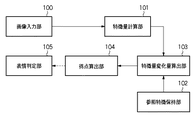•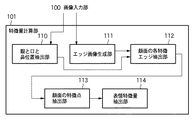•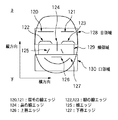•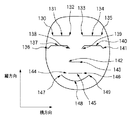•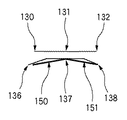•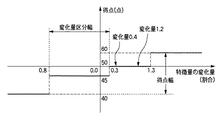•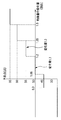•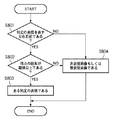•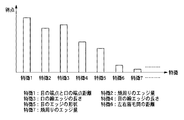••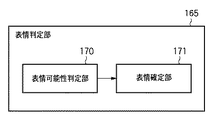•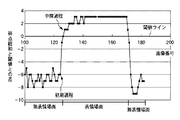•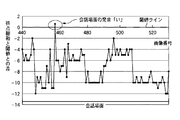•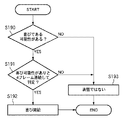•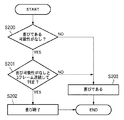•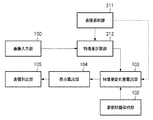•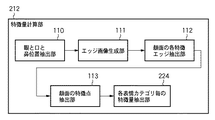•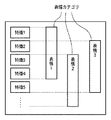•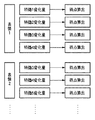•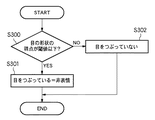•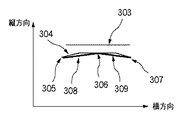•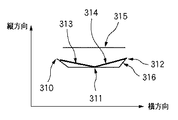•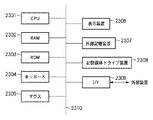•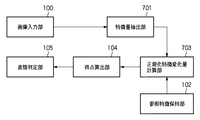•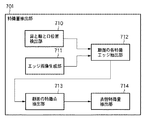•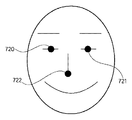•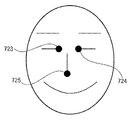•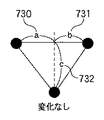•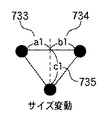•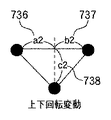•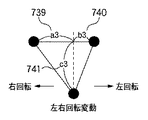•••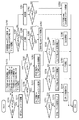•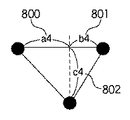••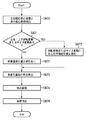•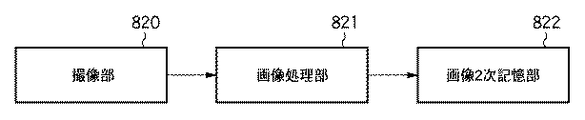•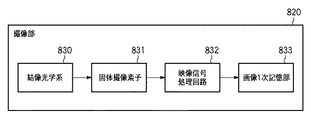•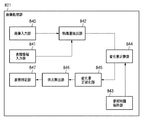•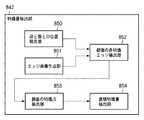•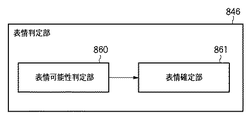•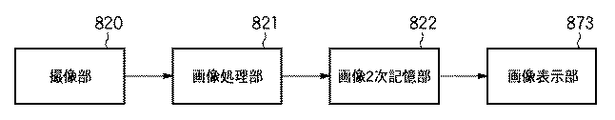## Abstract

PROBLEM TO BE SOLVED: To more accurately determine a facial expression in an image that is robust to individual differences and facial expressions. Furthermore, the facial expression in the image should be determined more accurately even when the face size changes or the face rotates.
An image input unit 100 inputs an image including a face, and a feature amount extraction unit 101 obtains a feature point for each of a predetermined region group in the face, and the face of an image including a face with a predetermined facial expression. A feature point is obtained for each of the predetermined region groups, and the normalized feature change amount calculation unit 103 obtains a change amount of each feature amount of the predetermined region group based on a difference between the respective feature amounts, and performs face size variation and face rotation. The change amount of each feature amount of the predetermined region group is normalized according to the variation, and the score calculation unit 104 calculates the score for each of the predetermined region group based on the change amount of each feature amount, and the facial expression determination unit 105 Is a comparison of the score distribution calculated for each of the predetermined region group and the distribution of the score for each of the predetermined region group calculated for each facial expression, so that the face of the face in the image input by the image input unit 100 is calculated. Judging facial expressions .
[Selection] Figure 1

## Description

The present invention relates to a technique for discriminating facial expressions in an input image.
As one of conventional facial expression recognition devices, a technique for determining emotion from facial expressions is disclosed (for example, see Patent Document 1). Emotion generally expresses emotions such as anger and sadness. According to the above technique, a predetermined facial expression element is extracted from each feature of the face based on related rules, and facial expression element information is extracted from the predetermined facial expression element. Extracting. Here, facial expression elements indicate eye opening / closing, eyebrow movement, forehead movement, lip up / down, lip opening / closing, and lower lip up / down. It consists of facial expression element information such as the tilt of the right eyebrow.
Next, a facial expression element code obtained by quantifying the facial expression element is calculated from facial expression element information constituting the obtained facial expression element based on a predetermined facial expression element quantification rule. Further, the emotion amount is calculated for each emotion category by a predetermined emotion conversion formula from a predetermined facial expression element code determined for each emotion category. Then, there is a method for determining the emotional maximum value in the emotion category as emotion.
Japanese Patent Publication No. 2573126
The shape and length of each facial feature varies greatly from person to person. For example, in an expressionless image that is a true face, it looks like a joy from a subjective point of view from a single image, such as a person with already lowered eyes or a person with narrow eyes, but it is a true face for the person himself / herself There is. Furthermore, the face size and orientation of the face image are not always constant, and if the face size changes or the face rotates, the features necessary for recognizing facial expressions are the face size changes and the face orientation. It is necessary to normalize according to the rotation fluctuation of the.
In addition, if the input image is a time-series image that assumes daily scenes including non-facial scenes that are conversational scenes in addition to facial expressions and faceless scenes that are face-to-face images, for example, surprised facial expressions There are cases where non-facial scenes such as pronunciations “O” in similar conversation scenes and pronunciations “i” and “e” similar to joyful expressions are misjudged as facial expressions.
The present invention has been made in view of the above problems, and an object thereof is to provide a technique for more accurately determining facial expressions in an image, which is robust to individual differences and facial expressions. It is another object of the present invention to provide a technique for accurately determining a facial expression even when the face size changes or the face rotates.
In order to achieve the object of the present invention, for example, an image processing apparatus of the present invention comprises the following arrangement.
That is, an input means for inputting an image including a face;
First feature amount calculating means for obtaining a feature amount for each of the predetermined region groups in the face in the image;
A second feature amount calculating means for obtaining a feature amount for each of the predetermined portion group of the face in an image including a face with a predetermined expression;
A change for obtaining a change amount of each feature amount of the predetermined region group based on a difference between the feature amount obtained by the first feature amount calculation unit and the feature amount obtained by the second feature amount calculation unit. A quantity calculation means;
Score calculating means for calculating a score for each of the predetermined part groups based on the amount of change obtained by the change amount calculating means for each of the predetermined part groups;
The input means inputs by comparing the score distribution calculated by the score calculation means for each of the predetermined part group with the distribution of scores for each of the predetermined part groups calculated for each facial expression. First judging means for judging the facial expression in the image.
In order to achieve the object of the present invention, for example, an image processing apparatus of the present invention comprises the following arrangement.
That is, an input means for inputting an image including a face;
First feature amount calculating means for obtaining a feature amount for each of the predetermined region groups in the face in the image;
A second feature amount calculating means for obtaining a feature amount for each of the predetermined part group of the face in an image including a face with a predetermined expression;
A change for obtaining a change amount of each feature amount of the predetermined part group based on a ratio between the feature amount obtained by the first feature amount calculation means and the feature amount obtained by the second feature amount calculation means. A quantity calculation means;
Score calculating means for calculating a score for each of the predetermined part groups based on the amount of change obtained by the change amount calculating means for each of the predetermined part groups;
The input means inputs by comparing the score distribution calculated by the score calculation means for each of the predetermined part group with the distribution of scores for each of the predetermined part groups calculated for each facial expression. First judging means for judging the facial expression in the image.
In order to achieve the object of the present invention, for example, an image processing apparatus of the present invention comprises the following arrangement.
That is, an input means for inputting an image including a face;
First feature amount calculating means for obtaining a feature amount for each of the predetermined region groups in the face in the image;
A second feature amount calculating means for obtaining a feature amount for each of the predetermined portion group of the face in an image including a face with a predetermined expression;
Based on the ratio between the feature quantity obtained by the first feature quantity calculation means and the feature quantity obtained by the second feature quantity calculation means, the change amount of each feature quantity of the predetermined region group was obtained. Then, a normalized feature change amount calculating means for normalizing a change amount of each feature amount of the predetermined region group by a normalized value based on a face size variation or a rotation variation,
Score calculating means for calculating a score for each of the predetermined part groups based on the amount of change obtained by the normalized feature change amount calculating means for each of the predetermined part groups;
The input means inputs by comparing the score distribution calculated by the score calculation means for each of the predetermined part group with the distribution of scores for each of the predetermined part groups calculated for each facial expression. First judging means for judging the facial expression in the image.
In order to achieve the object of the present invention, for example, an image processing apparatus of the present invention comprises the following arrangement.
That is, an input means for inputting an image including a face;
First feature amount calculating means for obtaining a feature amount for each of the predetermined region groups in the face in the image;
A second feature amount calculating means for obtaining a feature amount for each of the predetermined portion group of the face in an image including a face with a predetermined expression;
A change for obtaining a change amount of each feature amount of the predetermined part group based on a ratio between the feature amount obtained by the first feature amount calculation means and the feature amount obtained by the second feature amount calculation means. A quantity calculation means;
Change amount normalization that normalizes the change amount of each feature amount calculated by the change amount calculation means for obtaining the change amount of each feature amount of the predetermined part group by a normalization value based on face size variation or rotation variation Means,
Score calculating means for calculating a score for each of the predetermined part groups, based on the normalized amount of change obtained by the change amount normalizing part for each of the predetermined part groups;
The input means inputs by comparing the score distribution calculated by the score calculation means for each of the predetermined part group with the distribution of scores for each of the predetermined part groups calculated for each facial expression. First judging means for judging the facial expression in the image.
In order to achieve the object of the present invention, for example, an image processing apparatus of the present invention comprises the following arrangement.
That is, an input means for inputting an image including a face;
Facial expression information input means for inputting information indicating one or more facial expressions;
A first feature amount calculating means for performing a process for obtaining a feature amount for each of the predetermined part groups corresponding to the facial expression in the face in the image;
A second feature amount calculating means for obtaining a feature amount for each of the predetermined portion group of the face in an image including a face with a predetermined expression;
A change for obtaining a change amount of each feature amount of the predetermined region group based on a difference between the feature amount obtained by the first feature amount calculation unit and the feature amount obtained by the second feature amount calculation unit. A quantity calculation means;
Score calculating means for calculating a score for each of the predetermined part groups based on the amount of change obtained by the change amount calculating means for each of the predetermined part groups;
Judgment means for obtaining a sum value for each facial expression of the score calculated by the score calculation means for each of the predetermined part group, and judging the facial expression having the highest sum value as the facial expression in the image input by the input means It is characterized by including these.
In order to achieve the object of the present invention, for example, an image processing apparatus of the present invention comprises the following arrangement.
That is, an input means for inputting an image including a face;
Facial expression information input means for inputting information indicating one or more facial expressions;
A first feature amount calculating means for performing a process for obtaining a feature amount for each of the predetermined part groups corresponding to the facial expression in the face in the image;
A second feature amount calculating means for obtaining a feature amount for each of the predetermined portion group of the face in an image including a face with a predetermined expression;
A change for obtaining a change amount of each feature amount of the predetermined part group based on a ratio between the feature amount obtained by the first feature amount calculation means and the feature amount obtained by the second feature amount calculation means. A quantity calculation means;
Score calculating means for calculating a score for each of the predetermined part groups based on the amount of change obtained by the change amount calculating means for each of the predetermined part groups;
Judgment means for obtaining a sum value for each facial expression of the score calculated by the score calculation means for each of the predetermined part group, and judging the facial expression having the highest sum value as the facial expression in the image input by the input means It is characterized by including these.
In order to achieve the object of the present invention, for example, an image processing apparatus of the present invention comprises the following arrangement.
That is, an input means for inputting an image including a face;
Facial expression information input means for inputting information indicating one or more facial expressions;
A first feature amount calculating means for performing a process for obtaining a feature amount for each of the predetermined part groups corresponding to the facial expression in the face in the image;
A second feature amount calculating means for obtaining a feature amount for each of the predetermined portion group of the face in an image including a face with a predetermined expression;
Based on the ratio between the feature quantity obtained by the first feature quantity calculation means and the feature quantity obtained by the second feature quantity calculation means, the change amount of each feature quantity of the predetermined region group was obtained. Then, a normalized feature change amount calculating means for normalizing a change amount of each feature amount of the predetermined region group by a normalized value based on a face size variation or a rotation variation,
Score calculating means for calculating a score for each of the predetermined part groups based on the amount of change obtained by the normalized feature change amount calculating means for each of the predetermined part groups;
Judgment means for obtaining a sum value for each facial expression of the score calculated by the score calculation means for each of the predetermined part group, and judging the facial expression having the highest sum value as the facial expression in the image input by the input means It is characterized by including these.
In order to achieve the object of the present invention, for example, an image processing apparatus of the present invention comprises the following arrangement.
That is, an input means for inputting an image including a face;
Facial expression information input means for inputting information indicating one or more facial expressions;
A first feature amount calculating means for performing a process for obtaining a feature amount for each of the predetermined part groups corresponding to the facial expression in the face in the image;
A second feature amount calculating means for obtaining a feature amount for each of the predetermined portion group of the face in an image including a face with a predetermined expression;
A change for obtaining a change amount of each feature amount of the predetermined part group based on a ratio between the feature amount obtained by the first feature amount calculation means and the feature amount obtained by the second feature amount calculation means. A quantity calculation means;
Change in feature value that normalizes the change amount of each feature amount calculated by the change amount calculation means for obtaining the change amount of each feature amount of the predetermined part group by a normalized value based on face size variation or rotation variation A quantity normalization means;
Score calculating means for calculating a score for each of the predetermined part groups, based on the normalized amount of change obtained by the change amount normalizing part for each of the predetermined part groups;
Judgment means for obtaining a sum value for each facial expression of the score calculated by the score calculation means for each of the predetermined part group, and judging the facial expression having the highest sum value as the facial expression in the image input by the input means It is characterized by including these.
In order to achieve the object of the present invention, for example, an image processing method of the present invention comprises the following arrangement.
That is, an input process for inputting an image including a face;
A first feature amount calculating step for obtaining a feature amount for each of the predetermined region groups in the face in the image;
A second feature amount calculating step for obtaining a feature amount for each of the predetermined portion group of the face in an image including a face of a predetermined expression;
A change for obtaining a change amount of each feature amount of the predetermined part group based on a difference between the feature amount obtained in the first feature amount calculation step and the feature amount obtained in the second feature amount calculation step. A quantity calculation process;
Based on the amount of change obtained in the change amount calculation step for each of the predetermined portion groups, a score calculation step for calculating a score for each of the predetermined portion groups;
By comparing the distribution of the score calculated for each of the predetermined region group in the score calculation step with the distribution of the score for each of the predetermined region group calculated for each facial expression, the input was performed in the input step. And a first determination step of determining a facial expression in the image.
In order to achieve the object of the present invention, for example, an image processing method of the present invention comprises the following arrangement.
That is, an input process for inputting an image including a face;
A first feature amount calculating step for obtaining a feature amount for each of the predetermined region groups in the face in the image;
A second feature amount calculating step for obtaining a feature amount for each of the predetermined portion group of the face in an image including a face of a predetermined expression;
A change for obtaining a change amount of each feature amount of the predetermined part group based on a ratio between the feature amount obtained in the first feature amount calculation step and the feature amount obtained in the second feature amount calculation step. A quantity calculation process;
Based on the amount of change obtained in the change amount calculation step for each of the predetermined portion groups, a score calculation step for calculating a score for each of the predetermined portion groups;
By comparing the distribution of the score calculated for each of the predetermined region group in the score calculation step with the distribution of the score for each of the predetermined region group calculated for each facial expression, the input was performed in the input step. And a first determination step of determining a facial expression in the image.
In order to achieve the object of the present invention, for example, an image processing method of the present invention comprises the following arrangement.
That is, an input process for inputting an image including a face;
A first feature amount calculating step for obtaining a feature amount for each of the predetermined region groups in the face in the image;
A second feature amount calculating step for obtaining a feature amount for each of the predetermined portion group of the face in an image including a face of a predetermined expression;
Based on the ratio between the feature amount obtained in the first feature amount calculation step and the feature amount obtained in the second feature amount calculation step, the change amount of each feature amount of the predetermined part group was obtained. Thereafter, a normalized feature change amount calculation step of normalizing a change amount of each feature amount of the predetermined region group by a normalized value based on face size variation and rotation variation,
A score calculation step for calculating a score for each of the predetermined region groups based on the amount of change obtained in the normalized feature change amount calculation step for each of the predetermined region groups;
By comparing the distribution of the score calculated for each of the predetermined region group in the score calculation step with the distribution of the score for each of the predetermined region group calculated for each facial expression, the input was performed in the input step. And a first determination step of determining a facial expression in the image.
In order to achieve the object of the present invention, for example, an image processing method of the present invention comprises the following arrangement.
That is, an input process for inputting an image including a face;
A first feature amount calculating step for obtaining a feature amount for each of the predetermined region groups in the face in the image;
A second feature amount calculating step for obtaining a feature amount for each of the predetermined portion group of the face in an image including a face of a predetermined expression;
A change for obtaining a change amount of each feature amount of the predetermined part group based on a ratio between the feature amount obtained in the first feature amount calculation step and the feature amount obtained in the second feature amount calculation step. A quantity calculation process;
Change amount normalization that normalizes the change amount of each feature amount calculated in the change amount calculation step for obtaining the change amount of each feature amount of the predetermined part group by a normalization value based on face size variation or rotation variation Process,
A score calculation step of calculating a score for each of the predetermined region groups based on the normalized variation obtained in the variation amount normalization step for each of the predetermined region groups;
By comparing the distribution of the score calculated for each of the predetermined region group in the score calculation step with the distribution of the score for each of the predetermined region group calculated for each facial expression, the input was performed in the input step. And a first determination step of determining a facial expression in the image.
In order to achieve the object of the present invention, for example, an image processing method of the present invention comprises the following arrangement.
That is, an input process for inputting an image including a face;
A facial expression information input step for inputting information indicating one or more facial expressions;
A first feature amount calculation step of performing a process for obtaining a feature amount for each of the predetermined part groups corresponding to the facial expression in the face in the image;
A second feature amount calculating step for obtaining a feature amount for each of the predetermined portion group of the face in an image including a face of a predetermined expression;
A change for obtaining a change amount of each feature amount of the predetermined part group based on a difference between the feature amount obtained in the first feature amount calculation step and the feature amount obtained in the second feature amount calculation step. A quantity calculation process;
Based on the amount of change obtained in the change amount calculation step for each of the predetermined portion groups, a score calculation step for calculating a score for each of the predetermined portion groups;
A determination step of obtaining a sum value for each facial expression of the score calculated for each of the predetermined region groups in the score calculation step, and determining a facial expression having the highest total value as a facial expression in the image input in the input step It is characterized by including these.
In order to achieve the object of the present invention, for example, an image processing method of the present invention comprises the following arrangement.
That is, an input process for inputting an image including a face;
A facial expression information input step for inputting information indicating one or more facial expressions;
A first feature amount calculation step of performing a process for obtaining a feature amount for each of the predetermined part groups corresponding to the facial expression in the face in the image;
A second feature amount calculating step for obtaining a feature amount for each of the predetermined portion group of the face in an image including a face of a predetermined expression;
A change for obtaining a change amount of each feature amount of the predetermined part group based on a ratio between the feature amount obtained in the first feature amount calculation step and the feature amount obtained in the second feature amount calculation step. A quantity calculation process;
Based on the amount of change obtained in the change amount calculation step for each of the predetermined portion groups, a score calculation step for calculating a score for each of the predetermined portion groups;
A determination step of obtaining a sum value for each facial expression of the score calculated for each of the predetermined region groups in the score calculation step, and determining a facial expression having the highest total value as a facial expression in the image input in the input step It is characterized by including these.
In order to achieve the object of the present invention, for example, an image processing method of the present invention comprises the following arrangement.
That is, an input process for inputting an image including a face;
A facial expression information input step for inputting information indicating one or more facial expressions;
A first feature amount calculation step of performing a process for obtaining a feature amount for each of the predetermined part groups corresponding to the facial expression in the face in the image;
A second feature amount calculating step for obtaining a feature amount for each of the predetermined portion group of the face in an image including a face of a predetermined expression;
Based on the ratio between the feature amount obtained in the first feature amount calculation step and the feature amount obtained in the second feature amount calculation step, the change amount of each feature amount of the predetermined part group was obtained. Thereafter, a normalized feature change amount calculation step of normalizing a change amount of each feature amount of the predetermined region group by a normalized value based on face size variation and rotation variation,
A score calculation step for calculating a score for each of the predetermined region groups based on the amount of change obtained in the normalized feature change amount calculation step for each of the predetermined region groups;
A determination step of obtaining a sum value for each facial expression of the score calculated for each of the predetermined region groups in the score calculation step, and determining a facial expression having the highest total value as a facial expression in the image input in the input step It is characterized by including these.
In order to achieve the object of the present invention, for example, an image processing method of the present invention comprises the following arrangement.
That is, an input process for inputting an image including a face;
A facial expression information input step for inputting information indicating one or more facial expressions;
A first feature amount calculation step of performing a process for obtaining a feature amount for each of the predetermined part groups corresponding to the facial expression in the face in the image;
A second feature amount calculating step for obtaining a feature amount for each of the predetermined portion group of the face in an image including a face of a predetermined expression;
A change for obtaining a change amount of each feature amount of the predetermined part group based on a ratio between the feature amount obtained in the first feature amount calculation step and the feature amount obtained in the second feature amount calculation step. A quantity calculation process;
Change in feature value for normalizing the change amount of each feature amount calculated in the change amount calculation step for obtaining the change amount of each feature amount of the predetermined part group by a normalization value based on face size variation or rotation variation A quantity normalization process;
A score calculation step of calculating a score for each of the predetermined region groups based on the normalized variation obtained in the variation amount normalization step for each of the predetermined region groups;
A determination step of obtaining a sum value for each facial expression of the score calculated for each of the predetermined region groups in the score calculation step, and determining a facial expression having the highest total value as a facial expression in the image input in the input step It is characterized by including these.
In order to achieve the object of the present invention, for example, an imaging apparatus of the present invention comprises the following arrangement.
That is, imaging means,
An input means for inputting an image including a face;
First feature amount calculating means for obtaining a feature amount for each of the predetermined region groups in the face in the image;
A second feature amount calculating means for obtaining a feature amount for each of the predetermined portion group of the face in an image including a face with a predetermined expression;
A change for obtaining a change amount of each feature amount of the predetermined part group based on a ratio between the feature amount obtained by the first feature amount calculation means and the feature amount obtained by the second feature amount calculation means. A quantity calculation means;
Score calculating means for calculating a score for each of the predetermined part groups based on the amount of change obtained by the change amount calculating means for each of the predetermined part groups;
The input means inputs by comparing the score distribution calculated by the score calculation means for each of the predetermined part group with the distribution of scores for each of the predetermined part groups calculated for each facial expression. First judging means for judging facial expressions in the image;
Storage means for storing the image determined by the first determination means.
In order to achieve the object of the present invention, for example, an imaging apparatus of the present invention comprises the following arrangement.
That is, imaging means,
An input means for inputting an image including a face;
First feature amount calculating means for obtaining a feature amount for each of the predetermined region groups in the face in the image;
A second feature amount calculating means for obtaining a feature amount for each of the predetermined portion group of the face in an image including a face with a predetermined expression;
Based on the ratio between the feature quantity obtained by the first feature quantity calculation means and the feature quantity obtained by the second feature quantity calculation means, the change amount of each feature quantity of the predetermined region group was obtained. Then, a normalized feature change amount calculating means for normalizing a change amount of each feature amount of the predetermined region group by a normalized value based on a face size variation or a rotation variation,
Score calculating means for calculating a score for each of the predetermined part groups based on the normalized change amount obtained by the normalized feature change amount calculating means for each of the predetermined part groups;
The input means inputs by comparing the score distribution calculated by the score calculation means for each of the predetermined part group with the distribution of scores for each of the predetermined part groups calculated for each facial expression. First judging means for judging facial expressions in the image;
Storage means for storing the image determined by the first determination means.
In order to achieve the object of the present invention, for example, an imaging apparatus of the present invention comprises the following arrangement.
That is, imaging means,
An input means for inputting an image including a face;
First feature amount calculating means for obtaining a feature amount for each of the predetermined region groups in the face in the image;
A second feature amount calculating means for obtaining a feature amount for each of the predetermined portion group of the face in an image including a face with a predetermined expression;
A change for obtaining a change amount of each feature amount of the predetermined part group based on a ratio between the feature amount obtained by the first feature amount calculation means and the feature amount obtained by the second feature amount calculation means. A quantity calculation means;
Change in feature value that normalizes the change amount of each feature amount calculated by the change amount calculation means for obtaining the change amount of each feature amount of the predetermined part group by a normalized value based on face size variation or rotation variation A quantity normalization means;
Score calculating means for calculating a score for each of the predetermined part groups, based on the normalized amount of change obtained by the change amount normalizing part for each of the predetermined part groups;
The input means inputs by comparing the score distribution calculated by the score calculation means for each of the predetermined part group with the distribution of scores for each of the predetermined part groups calculated for each facial expression. First judging means for judging facial expressions in the image;
Storage means for storing the image determined by the first determination means.
In order to achieve the object of the present invention, for example, an imaging apparatus of the present invention comprises the following arrangement.
That is, imaging means,
An input means for inputting an image including a face;
Facial expression information input means for inputting information indicating one or more facial expressions;
A first feature amount calculating means for performing a process for obtaining a feature amount for each of the predetermined part groups corresponding to the facial expression in the face in the image;
A second feature amount calculating means for obtaining a feature amount for each of the predetermined portion group of the face in an image including a face with a predetermined expression;
A change for obtaining a change amount of each feature amount of the predetermined part group based on a ratio between the feature amount obtained by the first feature amount calculation means and the feature amount obtained by the second feature amount calculation means. A quantity calculation means;
Score calculating means for calculating a score for each of the predetermined part groups based on the amount of change obtained by the change amount calculating means for each of the predetermined part groups;
Judgment means for obtaining a sum value for each facial expression of the score calculated by the score calculation means for each of the predetermined part group, and judging the facial expression having the highest sum value as the facial expression in the image input by the input means When,
Storage means for storing the image determined by the determination means.
In order to achieve the object of the present invention, for example, an imaging apparatus of the present invention comprises the following arrangement.
That is, imaging means,
An input means for inputting an image including a face;
Facial expression information input means for inputting information indicating one or more facial expressions;
A first feature amount calculating means for performing a process for obtaining a feature amount for each of the predetermined part groups corresponding to the facial expression in the face in the image;
A second feature amount calculating means for obtaining a feature amount for each of the predetermined portion group of the face in an image including a face with a predetermined expression;
Based on the ratio between the feature quantity obtained by the first feature quantity calculation means and the feature quantity obtained by the second feature quantity calculation means, the change amount of each feature quantity of the predetermined region group was obtained. Then, a normalized feature change amount calculating means for normalizing a change amount of each feature amount of the predetermined region group by a normalized value based on a face size variation or a rotation variation,
Score calculating means for calculating a score for each of the predetermined part groups based on the amount of change obtained by the normalized feature change amount calculating means for each of the predetermined part groups;
Judgment means for obtaining a sum value for each facial expression of the score calculated by the score calculation means for each of the predetermined part group, and judging the facial expression having the highest sum value as the facial expression in the image input by the input means When,
Storage means for storing the image determined by the determination means.
In order to achieve the object of the present invention, for example, an imaging apparatus of the present invention comprises the following arrangement.
That is, imaging means,
An input means for inputting an image including a face;
Facial expression information input means for inputting information indicating one or more facial expressions;
A first feature amount calculating means for performing a process for obtaining a feature amount for each of the predetermined part groups corresponding to the facial expression in the face in the image;
A second feature amount calculating means for obtaining a feature amount for each of the predetermined portion group of the face in an image including a face with a predetermined expression;
A change for obtaining a change amount of each feature amount of the predetermined part group based on a ratio between the feature amount obtained by the first feature amount calculation means and the feature amount obtained by the second feature amount calculation means. A quantity calculation means;
Change in feature value that normalizes the change amount of each feature amount calculated by the change amount calculation means for obtaining the change amount of each feature amount of the predetermined part group by a normalized value based on face size variation or rotation variation A quantity normalization means;
Score calculating means for calculating a score for each of the predetermined part groups, based on the normalized amount of change obtained by the change amount normalizing part for each of the predetermined part groups;
Judgment means for obtaining a sum value for each facial expression of the score calculated by the score calculation means for each of the predetermined part group, and judging the facial expression having the highest sum value as the facial expression in the image input by the input means When,
Storage means for storing the image determined by the determination means.
In order to achieve the object of the present invention, for example, an imaging apparatus of the present invention comprises the following arrangement.
That is, imaging means,
An input means for inputting an image including a face;
Facial expression information input means for inputting information indicating one or more facial expressions;
First feature amount calculating means for obtaining a feature amount for each of the predetermined region groups in the face in the image;
A second feature amount calculating means for obtaining a feature amount for each of the predetermined portion group of the face in an image including a face with a predetermined expression;
A change for obtaining a change amount of each feature amount of the predetermined part group based on a ratio between the feature amount obtained by the first feature amount calculation means and the feature amount obtained by the second feature amount calculation means. A quantity calculation means;
Score calculating means for calculating a score for each of the predetermined part groups based on the amount of change obtained by the change amount calculating means for each of the predetermined part groups;
The score distribution calculated by the score calculation means for each of the predetermined part groups and the distribution of the scores for each of the predetermined part groups calculated for each facial expression of the facial expression information input by the facial expression information input means, A first determination means for determining a facial expression in the image input by the input means by comparing;
Storage means for storing the image determined by the first determination means.
In order to achieve the object of the present invention, for example, an imaging apparatus of the present invention comprises the following arrangement.
That is, imaging means,
An input means for inputting an image including a face;
Facial expression information input means for inputting information indicating one or more facial expressions;
First feature amount calculating means for obtaining a feature amount for each of the predetermined region groups in the face in the image;
A second feature amount calculating means for obtaining a feature amount for each of the predetermined portion group of the face in an image including a face with a predetermined expression;
Based on the ratio between the feature quantity obtained by the first feature quantity calculation means and the feature quantity obtained by the second feature quantity calculation means, the change amount of each feature quantity of the predetermined region group was obtained. Then, a normalized feature change amount calculating means for normalizing a change amount of each feature amount of the predetermined region group by a normalized value based on a face size variation or a rotation variation,
Score calculating means for calculating a score for each of the predetermined part groups based on the normalized change amount obtained by the normalized feature change amount calculating means for each of the predetermined part groups;
The score distribution calculated by the score calculation means for each of the predetermined part groups and the distribution of the scores for each of the predetermined part groups calculated for each facial expression of the facial expression information input by the facial expression information input means, A first determination means for determining a facial expression in the image input by the input means by comparing;
Storage means for storing the image determined by the first determination means.
In order to achieve the object of the present invention, for example, an imaging apparatus of the present invention comprises the following arrangement.
That is, imaging means,
An input means for inputting an image including a face;
Facial expression information input means for inputting information indicating one or more facial expressions;
First feature amount calculating means for obtaining a feature amount for each of the predetermined region groups in the face in the image;
A second feature amount calculating means for obtaining a feature amount for each of the predetermined portion group of the face in an image including a face with a predetermined expression;
A change for obtaining a change amount of each feature amount of the predetermined part group based on a ratio between the feature amount obtained by the first feature amount calculation means and the feature amount obtained by the second feature amount calculation means. A quantity calculation means;
Change in feature value that normalizes the change amount of each feature amount calculated by the change amount calculation means for obtaining the change amount of each feature amount of the predetermined part group by a normalized value based on face size variation or rotation variation A quantity normalization means;
Score calculating means for calculating a score for each of the predetermined part groups, based on the normalized amount of change obtained by the change amount normalizing part for each of the predetermined part groups;
The score distribution calculated by the score calculation means for each of the predetermined part groups and the distribution of the scores for each of the predetermined part groups calculated for each facial expression of the facial expression information input by the facial expression information input means, A first determination means for determining a facial expression in the image input by the input means by comparing;
Storage means for storing the image determined by the first determination means.
With the configuration of the present invention, it is possible to more accurately determine a facial expression in an image that is robust to individual differences and facial expressions. Furthermore, the facial expression in the image can be more accurately determined when the size of the face changes or when the face is rotated.
Hereinafter, the present invention will be described in detail according to preferred embodiments with reference to the accompanying drawings.
[First Embodiment]
FIG. 23 is a diagram illustrating a basic configuration of the image processing apparatus according to the present embodiment.
Reference numeral 2301 denotes a CPU that controls the entire apparatus using programs and data stored in the RAM 2302 and the ROM 2303 and also performs facial expression determination processing described later.
A RAM 2302 includes an area for storing programs and data loaded from the external storage device 307 and the storage medium drive device 2308, and also includes an area necessary for the CPU 2301 to perform various processes.
Reference numeral 2303 denotes a ROM that stores various programs such as a boot program and data.
Reference numerals 2304 and 2305 are a keyboard and a mouse, respectively, and can input various instructions to the CPU 2301.
A display device 2306 includes a CRT, a liquid crystal screen, and the like, and can display various information such as images and characters.
Reference numeral 2307 denotes an external storage device that functions as a large-capacity information storage device such as a hard disk drive device, and stores an OS (Operating System), a program relating to facial expression discrimination processing described later, and various data. These programs and data are read into the RAM 2302 according to instructions from the CPU 2301.
Reference numeral 2308 denotes a storage medium drive device that reads a program or data recorded on a storage medium such as a CD-ROM or DVD-ROM and outputs the read program or data to an external storage device 2307 or RAM 2302. It should be noted that a program, data, and the like related to a facial expression determination process described later may be recorded in this storage medium and read out to the RAM 2302 under the control of the CPU 2301 as necessary.
Reference numeral 2309 denotes an I / F for connecting the external apparatus and the apparatus, and data communication with the external apparatus is performed via the I / F.
Reference numeral 2310 denotes a bus connecting the above-described units.
FIG. 1 is a block diagram showing a functional configuration of the image processing apparatus according to the present embodiment.
The functional configuration of the image processing apparatus includes an image input unit 100 that inputs a plurality of images continuously in time series, and a feature amount necessary for discriminating a facial expression from an image (input image) input by the image input unit 100. A feature quantity calculation unit 101 that extracts a reference feature, a reference feature holding unit 102 that extracts and holds a reference feature necessary for recognizing an expression from a reference face of a true face (no expression) prepared in advance, and a feature A feature amount change amount for calculating a change amount of each feature amount of the face from the reference face by calculating a difference amount between the feature amount extracted by the amount calculation unit 101 and the feature amount held by the reference feature holding unit 102 The calculation unit 103, a score calculation unit 104 that calculates a score for each feature from the amount of change of each feature extracted by the feature amount change amount calculation unit 103, and an input image from the sum of the scores calculated by the score calculation unit 104 Face in Composed of facial expression determination unit 105 for determining the information.
1 may be configured by hardware. However, in this embodiment, the image input unit 100, the feature amount extraction unit 101, the feature amount change amount calculation unit 103, the score calculation unit 104, and the facial expression determination unit. Each unit 105 is configured by a program, and this program is stored in the RAM 2302, and is executed by the CPU 2301, thereby realizing the function of each unit. The reference feature holding unit 102 is a predetermined area in the RAM 2302, but may be an area in the external storage device 2307.
Below, each part shown in FIG. 1 is demonstrated in detail.
The image input unit 100 inputs a time-series face image obtained by cutting out a moving image obtained from a video camera or the like for each frame as an input image. That is, according to the configuration of FIG. 23, image data of each frame is sequentially output to the RAM 2302 via the I / F 2309 from a video camera or the like connected to the I / F 2309.
As shown in FIG. 2, the feature quantity extraction unit 101 includes an eye / mouth / nose position extraction unit 110, an edge image generation unit 111, facial feature edge extraction units 112, a facial feature point extraction unit 113, and facial expression feature quantity extraction. Part 114. FIG. 2 is a block diagram illustrating a functional configuration of the feature amount extraction unit 101.
Hereafter, each part shown in FIG. 2 is demonstrated in detail.
The eye, mouth, and nose position extraction unit 110 determines a predetermined part of the face, that is, the position of the eye, mouth, and nose (position in the input image) from the image (input image) input by the image input unit 100. The method for determining the position of the eyes and mouth is, for example, preparing eye, mouth, and nose templates, and performing template matching to extract eye, mouth, and nose candidates. A method of detecting the position of the eyes, mouth, and nose by using the spatial arrangement relationship between the candidates of the eyes, mouth, and nose obtained by the above and the skin color information that is color information can be used. The detected eye and mouth position data is output to each feature edge extraction unit 112 of the subsequent face.
Next, an edge image is extracted from the input image obtained by the image input unit 100 by the edge image generation unit 111, an edge expansion process is performed on the extracted edge, and then a thinning process is performed to generate an edge image. For example, edge extraction using a Sobel filter can be used for edge extraction, 8-neighbor expansion processing can be used for edge expansion processing, and Hilditch thinning processing can be used for thinning processing. Here, the edge expansion process and the thinning process are intended to smoothly perform edge scanning and feature point extraction, which will be described later, by connecting the split edges by expanding the edges and performing the thinning process. Yes. The generated edge image is output to each feature edge extraction unit 112 of the subsequent face.
Each facial feature edge extraction unit 112 uses the eye and mouth position data detected by the eye and mouth position extraction unit 110 and the edge image generated by the edge image generation unit 111 to generate an eye region in the edge image as shown in FIG. Determine cheek area and mouth area.
The eye area is set so that only the eyebrows and the eye edge are included in the area, the cheek area is set so that only the cheek edge and the nose edge are included, and the mouth area is the upper lip edge, the tooth edge, Specify the area so that only the lower lip edge is included.
Here, an example of setting processing of these areas will be described.
The vertical width of the eye area is 0.5 times the distance between the left and right eye position detection from the middle point of the left eye position detection result and the right eye position detection result obtained from the template matching and the spatial arrangement relationship, and the left eye position detection is below A region having a distance of 0.3 times the distance is defined as a vertical region of the eyes.
Regarding the lateral width of the eye region, the region of the distance between the left and right eye positions detected from the middle point of the left eye position detection result and the right eye position detection result obtained from the template matching and the spatial arrangement relationship is set as the lateral region of the eye.
That is, the length of the side of the eye region in the vertical direction is 0.8 times the distance between the left and right eye position detection, and the length of the side in the horizontal direction is twice the distance between the left and right eye position detection.
The vertical width of the mouth area is 0.75 times the distance between the nose position detection and the mouth detection position above the position of the mouth position detection result obtained from the template matching and the spatial arrangement relationship, and below the left eye position detection result and the right eye position. An area that is 0.25 times the distance between the midpoint of the detection result and the mouth position detection result is defined as a vertical area. The lateral width of the mouth area is 0.8 times the distance between the left and right eye positions detected from the position of the mouth position detection result obtained from the template matching and the spatial arrangement relationship.
The vertical width of the cheek area is above and below the midpoint of the left eye position detection result, right eye position detection result, and mouth position detection result obtained from template matching and spatial layout (the point near the center of the face) An area that is 0.25 times the distance between the midpoint of the left eye position detection result and the right eye position detection result and the mouth position detection result is defined as the vertical direction area.
The width of the cheek area is determined from the midpoint of the left eye position detection result, the right eye position detection result, and the mouth position detection result obtained from the template matching and the spatial arrangement relationship. An area that is 0.6 times the distance between the left and right eye position detection is defined as a lateral area of the cheek.
That is, the vertical side length of the cheek region is 0.5 times the distance between the midpoint of the left eye position detection result and the right eye position detection result and the mouth position detection result, and the horizontal side length is left and right. This is 1.2 times the distance between eye position detection.
With the above region setting process, as shown in FIG. 3, the first edge 120 and edge 121 from the top are determined as eyebrow edges, and the second edge 122 and edge 123 are determined as eye edges in the eye region. When the mouth is closed in the mouth area, as shown in FIG. 3, the first edge 126 from the top is determined as the upper lip edge, the second edge 127 is determined as the lower lip edge, and the mouth is opened. The first edge from the top is determined as the upper lip edge, the second edge as the tooth edge, and the third edge as the lower lip edge.
The above determination results are data indicating whether each of the above three regions (eye region, cheek region, mouth region) is an eye region, cheek region, or mouth region, and data on the position and size of each region. Are generated by the feature edge extraction unit 112 of the face, and output to the feature point extraction unit 113 of the face together with the edge image.
The facial feature point extraction unit 113 uses the various data input from the facial feature edge extraction unit 112 to scan the edges in the eye area, cheek area, and mouth area in the edge image, thereby performing each of the following. Detect feature points.
FIG. 4 is a diagram illustrating each feature point detected by the feature point extraction unit 113 of the face. As shown in the figure, each feature point is the end point of each edge and the midpoint between the end points on the edge. These are, for example, the values of the pixels constituting the edge (here, the values of the pixels constituting the edge). 1), the edge points of the edge can be obtained by obtaining the maximum value and the minimum value of the coordinate position in the horizontal direction, and between the end points on the edge. The midpoint can be obtained by simply setting the horizontal coordinate value of the midpoint between the end points on the edge.
The facial feature point extraction unit 113 obtains position information of these end points as feature point information, eye feature point information (position information of feature points of each edge in the eye region), mouth feature point information (each of the mouth regions) The edge feature point position information) is output to the subsequent facial expression feature quantity extraction unit 114 together with the edge image.
As for the feature points, a template for calculating the positions of the end points of the eyes, mouth, and nose may be used in the same manner as the eye / mouth / nose position detection, and the feature points are not limited to feature point extraction by edge scanning.
The facial expression feature amount extraction unit 114 determines from the feature point information obtained by the facial feature point extraction unit 113 the “edge density around the forehead”, “the shape of the eyebrows edge”, and “the eyebrow edge shape” necessary for facial expression discrimination. "Distance", "Distance between eyebrows edge and eye edge", "Distance between eye end point and mouth end point", "Length of eye line edge", "Shape of eye line edge", "Edge around cheek" Features such as “density”, “length of mouth line edge”, and “shape of mouth edge” are calculated.
Here, the “distance between the end point of the eye and the end point of the mouth” is the vertical direction from the coordinate position of the feature point 136 (right end point of the right eye) in FIG. 4 to the coordinate position of the feature point 147 (right end point of the lips). Similarly, the distance in the vertical direction from the coordinate position of the feature point 141 (left end point of the left eye) to the coordinate position of the feature point 149 (left end point of the lips).
Further, “the length of the eye line edge” is the distance in the horizontal direction from the coordinate position of the feature point 136 (right end point of the right eye) in FIG. 4 to the coordinate position of the feature point 138 (left end point of the right eye). Or the distance in the horizontal direction from the coordinate position of the feature point 139 (the right end point of the left eye) to the coordinate position of the feature point 141 (the left end point of the left eye).
Further, as shown in FIG. 5, the “shape of the eye line edge” is a line segment (straight line) defined by the feature point 136 (right end point of the right eye) and the feature point 137 (midpoint of the right eye). 150, a line segment (straight line) 151 defined by the feature point 137 (the middle point of the right eye) and the feature point 138 (the left end point of the right eye) is calculated, and the two straight lines 150 and 151 calculated The shape is determined from the inclination.
This process is the same as the process for obtaining the shape of the line edge of the left eye, and only the feature points used are different. That is, the slope of the line segment defined by the feature point 139 (the right end point of the left eye) and the feature point 140 (the midpoint of the left eye), the feature point 140 (the midpoint of the left eye), and the feature point 141 (the left eye) Is determined in the same manner.
Further, “edge density around cheek” represents the number of pixels constituting the edge in the cheek area. This is because wrinkles are generated when the muscles of the cheeks are lifted. As a result, various edges having different lengths and thicknesses are generated. As the amount of these edges, the number of pixels constituting these edges (pixels) The number of pixels having a value of 1) is counted, and the density can be obtained by dividing by the number of images constituting the cheek region.
Also, “the length of the mouth edge” means that all edges are scanned in the mouth area, and the pixel having the smallest horizontal coordinate position among the pixels constituting the edge is the feature point 147 (the right end point of the mouth). When the largest pixel is the feature point 149 (the left end point of the mouth), the distance from the coordinate position of the feature point 147 (the right end point of the mouth) to the coordinate position of the feature point 149 (the left end point of the mouth) is indicated. Is.
In addition, as described above, the distance between the end points, the slope of the line segment defined by the two end points, and the edge density are obtained in order to obtain the feature amount. Thus, the feature amount such as the length and shape of the image is obtained. Therefore, hereinafter, the length and shape of these edges may be collectively referred to as “edge feature amounts”.
As described above, the feature quantity extraction unit 101 can obtain each feature quantity from the input image.
Returning to FIG. 1, the reference feature holding unit 102 detects, in advance from the facial expression image that is a true face, by the feature amount detection process performed by the feature amount extraction unit 101 before performing the facial expression determination process. The feature amount in the expressionless face is retained.
Therefore, in the process described below, how much the feature quantity detected by the feature quantity extraction unit 101 from the edge image of the input image by the feature quantity detection process has changed from the feature quantity held by the reference feature holding unit 102. The facial expression in the input image is discriminated according to the amount of change. Accordingly, the feature quantity held by the reference feature holding unit 102 may be referred to as “reference feature quantity” hereinafter.
First, the feature amount change amount calculation unit 103 calculates a difference between the feature amount detected by the feature amount extraction unit 101 from the edge image of the input image by the feature amount detection process and the feature amount held by the reference feature holding unit 102. To do. For example, the “distance between the eye end point and the mouth end point” detected by the feature amount extracting unit 101 from the edge image of the input image by the feature amount detecting process, and the “eye end point and mouth end point” held by the reference feature holding unit 102. The difference from the “endpoint distance” is calculated, and the amount of change in the feature amount is calculated. Obtaining such a difference calculation for each feature amount, in other words, obtaining a change in the feature amount of each part.
When calculating the difference between the feature amount detected by the feature amount detection process from the edge image of the input image by the feature amount extraction unit 101 and the feature amount held by the reference feature holding unit 102, naturally, the same feature is used. Since the difference between the objects is taken (for example, “the distance between the eye end point and the mouth end point” detected by the feature amount extraction unit 101 from the edge image of the input image by the feature amount detection process, the reference feature holding unit 102 holds The calculation of the difference between the “distance between the eye end point and the mouth end point”) needs to be related to each other, but this method is not particularly limited.
Note that this reference feature amount may be greatly different for each user. In this case, even if this reference feature amount matches a certain user, it may not match another user. Accordingly, the reference feature holding unit 102 may store reference feature amounts of a plurality of users. In this case, in advance of inputting the image from the image input unit 100, information indicating who the face image is input is input in advance, and this is performed when the feature amount change amount calculation unit 103 performs processing. If the reference feature amount is determined based on the information, the difference can be calculated using the reference feature amount for each user, and the accuracy of facial expression determination processing described later can be further improved.
Further, the reference feature holding unit 102 detects this feature amount detection process performed by the feature amount extraction unit 101 from an expressionless image on the average face instead of the reference feature amount for each user. The feature amount in the expressionless face may be held.
In this way, the data of each change amount indicating the change in the feature amount of each part obtained by the feature amount change amount calculation unit 103 is output to the score calculation unit 104 in the subsequent stage.
The score calculation unit 104 calculates a score based on the amount of change of each feature value and the “weight” obtained in advance and held in a memory (for example, the RAM 2302). As for the weight, an analysis is performed in advance for individual differences in the amount of change for each part, and an appropriate weight is set for each feature amount according to the analysis result.
For example, features with relatively small changes, such as the length of the edge of the eye, and features with individual differences in the amount of change, such as wrinkles, are weighted less. It is to take a large weight.
FIG. 6 is a graph that is referred to in order to calculate a score from the amount of change in eye edge length, which is a feature in which there is an individual difference in the amount of change.
The horizontal axis represents a feature amount change amount (hereinafter, a value normalized by the feature amount in the reference face), and the vertical axis represents a score. For example, the amount of change in the length of the eye edge is 0.4. Then, the score is calculated as 50 points from the graph. Even when the amount of change in the length of the eye edge is 1.2, the score is calculated as 50 points in the same way as when the amount of change is 0.3, and even if the amount of change varies greatly depending on individual differences, Is weighted so as to be small.
FIG. 7 is a graph that is referred to in order to calculate a score from the amount of change in the length of the distance between the end points of the eyes and the mouth, which is a feature that does not cause individual differences in the amount of change.
As in FIG. 6, the horizontal axis represents the amount of change in the feature amount, and the vertical axis represents the score. For example, when the amount of change in the length of the end point distance between the eyes and the mouth is 1.1, 50 points are obtained from the graph. Is calculated, and 55 points are calculated from the graph when the amount of change in the length of the end point distance between the eyes and the mouth is 1.3. That is, weighting is performed so that the score difference becomes large when the amount of change greatly varies depending on individual differences.
That is, the “weight” corresponds to the ratio between the change amount segment width and the score width when the score calculation unit 104 calculates the score. By performing the process of setting the weight for each feature amount in this way, the individual difference in the feature amount change amount is absorbed, and the facial expression discrimination does not depend on only one feature, so that false detection and non-detection are reduced. The facial expression discrimination (recognition) rate can be improved.
The RAM 2302 stores the data of the graphs shown in FIGS. 5 and 6, that is, data indicating the correspondence between the amount of change in the feature amount and the score, and the score is calculated using this data.
The score data for each feature amount obtained by the score calculation unit 104 is output to the subsequent facial expression determination unit 105 together with data indicating which feature amount each score is for.
The RAM 2302 stores score data for each feature amount obtained by the above processing by the score calculation unit 104 in advance for each facial expression before performing facial expression discrimination processing.
Therefore, the facial expression determination unit 105
1 Comparing processing of the total value of scores for each feature amount and a predetermined threshold value 2 By performing processing for comparing the distribution of scores for each feature amount and the distribution of scores for each feature amount for each facial expression And facial expression discrimination.
For example, the expression of joy is as follows: 1 The bottom of the eye is lowered 2 The muscles of the cheeks are lifted 3 The edge of the mouth is lifted, and the distribution of the calculated scores is as shown in FIG. Score of “distance between mouth end point”, “edge density around cheek”, and “line edge length of mouth” are very high, followed by “line edge length of eye”, “line edge of eye” The score of “shape” is also higher than other features, and the score distribution is unique to joyful expressions. This unique score distribution is the same for other facial expressions. FIG. 9 is a diagram showing the distribution of scores for the expression of joy.
Therefore, the facial expression determination unit 105 identifies the shape of the distribution based on the score of each feature amount obtained by the score calculation unit 104 is closest to the shape of the score distribution specific to which facial expression, and the score distribution of the closest shape is The facial expression shown is the facial expression to be output as the determination result.
Here, as a method of finding the score distribution with the closest shape, for example, the distribution shape is approximated to a mixed Gaussian and parametrically modeled, and similarity determination between the obtained score distribution and the score distribution provided for each facial expression is performed. Is determined by determining the magnitude of the distance in the parameter space. Then, the facial expression indicated by the score distribution (score distribution with a smaller distance) having a higher degree of similarity to the obtained score distribution is set as a candidate for determination.
Next, a process of determining whether or not the total score of each feature amount obtained by the score calculation unit 104 is equal to or greater than a threshold value is performed. This comparison process is a more effective process for accurately determining a non-expression scene similar to an expression scene as an expression scene. Therefore, if the total value is equal to or greater than a predetermined threshold, the candidate is determined as the finally determined facial expression. On the other hand, if the total value is smaller than a predetermined threshold, the candidate is discarded and it is determined that the face in the input image is expressionless or non-expression.
In the comparison processing of the shape of the score distribution, if the similarity is not more than a certain value, it is determined that the face in the input image is no expression or no expression at this time, and the score calculation unit 104 obtains it. Alternatively, the process may be terminated without performing the comparison process between the total value of the score of each feature amount and the threshold value.
FIG. 8 is a flowchart of a determination process when it is determined whether or not the facial expression in the input image is a “specific expression” using the score for each feature amount obtained by the score calculation unit 104.
First, the facial expression determination unit 105 determines whether or not the shape of the distribution of each feature value obtained by the score calculation unit 104 is close to the shape of the score distribution specific to a specific facial expression (step S801). For example, if the degree of similarity between the obtained score distribution and the score distribution of a specific facial expression is equal to or greater than a predetermined value, “the shape of the distribution by the score of each feature amount obtained by the score calculation unit 104 is a specific value. It is determined that it is close to the shape of the score distribution peculiar to facial expressions.
If it is determined that the points are close, the process proceeds to step S802. Next, a determination process is performed to determine whether or not the total value of the scores of the respective feature values obtained by the score calculation unit 104 is equal to or greater than a predetermined threshold (step). S802). If it is determined that the value is greater than or equal to the threshold value, it is determined that the facial expression in the input image is the “specific expression”, and the determination result is output.
On the other hand, if it is determined in step S801 that it is not close, or if it is determined in step S802 that the total value is smaller than the threshold value, the process proceeds to step S804, and the input image is a non-expression image or no expression. Data indicating that the image is an image is output (step S804).
In the present embodiment, as facial expression discrimination processing, the comparison between the total value of scores for each feature amount and a predetermined threshold, the distribution of scores for each feature amount, and the score for each feature amount for each facial expression However, the present invention is not limited to this, and only one of the comparison processes may be performed.
With the above processing, according to the present embodiment, the comparison processing of score distribution and the comparison processing with the total value of the scores are performed, so it is possible to more accurately determine which facial expression is the facial expression in the input image. It is also possible to determine whether the facial expression in the input image is a specific facial expression.
[Second Embodiment]
FIG. 10 is a block diagram showing a functional configuration of the image processing apparatus according to the present embodiment. The same parts as those in FIG. 1 are denoted by the same reference numerals, and the description thereof is omitted. The basic configuration of the image processing apparatus according to this embodiment is the same as that of the first embodiment, that is, the same as that shown in FIG.
The image processing apparatus according to this embodiment will be described below. As described above, the functional configuration of the image processing apparatus according to the present embodiment is different from the functional configuration of the image processing apparatus according to the first embodiment in the facial expression determination unit 165. Therefore, the facial expression determination unit 165 will be described in detail below.
FIG. 11 is a block diagram illustrating a functional configuration of the facial expression determination unit 165. As shown in the figure, the facial expression determination unit 165 includes a facial expression possibility determination unit 170 and a facial expression determination unit 171.
The facial expression possibility determination unit 170 performs facial expression determination processing similar to that of the first embodiment using the score distribution composed of the score of each feature value obtained from the score calculation unit 104 and the total value of each score. The determination result is “expression possibility determination result”. For example, when determining whether or not the expression is a joyful expression, instead of determining that the expression is a joyful expression from the distribution of the scores obtained by the score calculation unit 104 and the total value, It may be a facial expression ”.
This possibility determination is made, for example, because each feature change of the pronunciation “I” and “E” in the conversation scene which is a non-expression scene is almost the same feature change as each feature change of the face of the joy scene. This is done to distinguish these non-facial scenes and joyful scenes.
Next, the facial expression determination unit 171 uses the facial expression possibility determination result obtained by the facial expression possibility determination unit 170 to determine that it is a specific facial expression image. In FIG. 12, the horizontal axis represents the image number uniquely assigned to each time series image, and the vertical axis represents the difference between the sum of the points and the threshold line 182, and the total score when the faceless expression scene changes from a true face to a joy expression scene. FIG. 6 is a diagram illustrating a difference between a threshold line and a threshold line.
In FIG. 13, the horizontal axis represents the image number of the time-series image, the vertical axis represents the difference between the sum of the scores and the threshold line 183, and the difference between the score sum of the conversation scene that is a non-facial expression scene and the threshold line 183 is shown. is there.
Referring to the case of changing from an expressionless scene to a joyful expression scene in FIG. 12, the score change from the initial process to the intermediate process varies greatly, but after passing the intermediate process, the score change becomes gradual. The score is almost constant. In other words, each part of the face such as the eyes and mouth of the face changes suddenly from the initial process to the joyful expression scene from the expressionless scene to the joyful expression scene. This shows that the fluctuations in the figure are gradual and will eventually cease.
It can be said that the variation characteristic of each feature of the face is the same for other facial expressions. Conversely, referring to the conversation scene that is a non-facial expression scene in FIG. 13, there is an image whose score exceeds the threshold line in the conversation scene of pronunciation “I” in which each feature change of the face of the eyes and mouth is almost the same as pleasure. To do. However, unlike the joy expression scene in the conversation scene of pronunciation “I”, each feature of the face is constantly changing rapidly, so even if the score exceeds the threshold line, the score immediately becomes the threshold line. There is a tendency to become as follows.
Therefore, the possibility of facial expression is determined by the expression possibility determination unit 170, and the conversation scene and the expression scene are more accurately performed by performing a step of determining the expression by the expression determination unit 171 from the continuity of the expression possibility determination result. Can be determined.
In the visual psychological research on the recognition of facial expressions by humans, it has been found that the movement of the face, especially the speed, is a factor that determines the emotion category judgment from the facial expression. Kamachi, V .; Bruce, S.M. Mukaida, J. et al. Gyoba, S .; Yoshikawa, and S.J. Akamatsu, "Dynamic properties influencing the perception of facial expression," Perception, vol. 30, pp. 875-887, July 2001.
Next, processing performed by the facial expression possibility determination unit 170 and the facial expression determination unit will be described in more detail.
First, it is assumed that the possibility determination unit 170 determines that the input image (the mth frame image) is “the first facial expression”. This determination result is output to the facial expression determination unit 171 as a possibility determination result. The facial expression determination unit 171 does not output this determination result immediately, but instead counts the number of times that the possibility determination unit 170 determines that it is the first facial expression. This count is reset to 0 when the possibility determination unit 170 determines that the second facial expression is different from the first facial expression.
The facial expression determination unit 171 does not immediately output the facial expression determination result (the determination result that the facial expression is the first facial expression) as described above. This is because the facial expression determined here is still unsatisfactory due to the various factors described above. This is because it may be clear.
The possibility determination unit 170 continues to perform facial expression determination processing for each input image, such as the input image of the (m + 1) th frame and the input image of the (m + 2) th frame, but the facial expression determination unit 171 When the count value of n reaches n, that is, when the possibility determination unit 170 determines that all the n frames are continuously “first facial expression” from the mth frame, the facial expression determination unit 171 determines that “ Data indicating that “the start of the first facial expression”, that is, the (m + n) th frame is the start frame is recorded in the RAM 2302, and after this point, the possibility determination unit 170 is different from the first facial expression. The expression up to the point when the second expression is determined is determined as a joy expression.
As described above with reference to FIG. 12, in the facial expression scene, the difference between the score total for a certain period and the threshold value does not change, that is, the same expression continues for a certain period. Conversely, if the same facial expression does not continue for a certain period, there is a possibility of a conversation scene that is a non-facial scene as described above with reference to FIG.
Accordingly, if the possibility of the same facial expression is determined for a certain period (here, n frames) by the above processing performed by the possibility determination unit 170, the facial expression is output as a final determination result for the first time. Disturbing elements can be removed from the facial expression determination process in a conversation scene or the like, which is a facial expression scene, and a more accurate facial expression determination process can be performed.
FIG. 14 is a flowchart of processing performed by the facial expression determination unit 171 to determine the start time of a joyful facial expression in images continuously input from the image input unit 100.
First, when the possibility determination result by the possibility determination unit 170 indicates that the user is happy (step S190), the process proceeds to step S191, and the count value by the facial expression determination unit 171 is p (FIG. 14). P = 4) (step S191), that is, when the possibility determination result by the possibility determination unit 170 is determined to be pleasure for p frames consecutively, this point of time is referred to as “joy start”. Judgment is made, and data indicating this (for example, current frame number data and flag data indicating pleasure start) is recorded in the RAM 2302 (step S192).
Through the above processing, the start time (start frame) of the expression of joy can be specified.
FIG. 15 is a flowchart of processing performed by the facial expression determination unit 171 to determine the end time of a joyful facial expression in images continuously input from the image input unit 100.
First, the facial expression determination unit 171 refers to the flag data recorded in the RAM 2302 in step S192, and determines whether the expression of joy is currently started or not ended (step S200). As will be described later, when the expression of joy ends, this data is rewritten to that effect, so it is possible to determine whether or not the expression of joy is currently ended by referring to this data.
If the expression of joy has not ended yet, the process proceeds to step S201, and the expression possibility unit 170 determines that there is no possibility of joy q (q = 3 in FIG. 15) frames continuously. (When the count by the facial expression determination section 171 is q frame continuous 0), this point is determined as “joy end”, and the flag data is rewritten to “data indicating that pleasure ends” and recorded in the RAM 2302 (Step S202).
However, in step S201, if it is not determined that there is no possibility that the expression possibility unit 170 is delighted in q frames continuously (when the count by the expression determination unit 171 is not q frames continuous 0), the final determination is made. As a facial expression determination result, the facial expression in the input image is determined to be “happy”, and the data is not manipulated.
After the expression of joy ends, the expression determination unit 171 determines that the expression in each frame from the start to the end is “joy”.
In this way, the expression start image and the expression end image are determined, and all the images between them are determined to be expression images, thereby suppressing the occurrence of misjudgment in the expression determination process for the images in between. As a whole, the accuracy of facial expression determination processing can be improved.
In this embodiment, the processing for determining the expression of “joy” has been described as an example. However, even if this expression is other than “joy”, the processing contents are basically the same. is there.
[Third Embodiment]
FIG. 16 is a block diagram illustrating a functional configuration of the image processing apparatus according to the present embodiment. Parts that perform substantially the same operations as in FIG. 1 are given the same numbers, and descriptions thereof are omitted. The basic configuration of the image processing apparatus according to this embodiment is the same as that of the first embodiment, that is, the same as that shown in FIG.
The image processing apparatus according to the present embodiment inputs one or more candidates for the facial expression in the input image, and the facial expression in the input image is any one of the input ones or more. This is a judgment.
Hereinafter, the image processing apparatus according to the present embodiment will be described in more detail. As described above, the functional configuration of the image processing apparatus according to the present embodiment differs from the functional configuration of the image processing apparatus according to the first embodiment in that the facial expression selection unit 211, the feature amount extraction unit 212, and the facial expression determination unit. 216. Therefore, the facial expression selection unit 211, the feature amount extraction unit 212, and the facial expression determination unit 216 will be described in detail below.
The facial expression selection unit 211 is for inputting one or more facial expression candidates. For input, for example, one or more facial expressions may be selected using a keyboard 2304 or a mouse 2305 on a GUI for selecting a plurality of facial expressions displayed on the display screen of the display device 2306. The selected result is output to the feature amount extraction unit 212 and the feature amount change amount calculation unit 103 as a code (for example, a number).
The feature amount extraction unit 212 performs processing for obtaining a feature amount for recognizing the facial expression selected by the facial expression selection unit 211 from the face in the image input from the image input unit 100.
The facial expression determination unit 216 performs a process of determining which face in the image input from the image input unit 100 is the facial expression selected by the facial expression selection unit 211.
FIG. 17 is a block diagram illustrating a functional configuration of the feature amount extraction unit 212. In the figure, the same parts as those in FIG. 2 are denoted by the same reference numerals, and the description thereof is omitted. Hereinafter, each unit illustrated in FIG. 17 will be described.
The feature amount extraction unit 224 for each facial expression uses the feature point information obtained by the facial feature point extraction unit 113 to calculate a feature amount according to the facial expression selected by the facial expression selection unit 211.
FIG. 18 is a diagram showing feature amounts corresponding to the facial expressions (expression 1, facial expression 2, facial expression 3) selected by the facial expression selection unit 211. FIG. For example, according to the figure, it is necessary to calculate features 1 to 4 to recognize facial expression 1, and to calculate features 2 to 5 to recognize facial expression 3.
For example, assuming that the joy expression is selected by the expression selection unit 211, the features required for the joy expression are the distance between the end points of the eyes and the mouth, the length of the eye edge, the inclination of the eye edge, the length of the mouth edge, Individual feature amounts are required for each facial expression, such as edge inclination and edge density around the cheek.
Such a table (characteristic table shown in FIG. 18) indicating the feature amount necessary for recognizing each facial expression, that is, a code indicating the facial expression input from the facial expression selection unit 211, It is assumed that a table that is associated with data indicating what kind of feature value is obtained in order to recognize a facial expression is recorded in the RAM 2302 in advance.
As described above, since the code corresponding to the selected facial expression is input from the facial expression selection unit 211, the feature amount extraction unit 212 refers to this table to recognize the facial expression corresponding to this code. The amount can be specified, and as a result, the feature amount corresponding to the facial expression selected by the facial expression selection unit 211 can be calculated.
Returning to FIG. 16, the subsequent feature amount change amount calculation unit 103 calculates the difference between the feature amount by the feature amount extraction unit 212 and the feature amount held by the reference feature holding unit 102 as in the first embodiment. calculate.
Note that the number and type of feature amounts calculated by the feature amount extraction unit 212 differ depending on facial expressions. Therefore, the feature amount change amount calculation unit 103 according to the present embodiment reads out and uses the feature amount necessary for recognizing the facial expression selected by the facial expression selection unit 211 from the reference feature holding unit 102. The feature amount necessary for recognizing the facial expression selected by the facial expression selection unit 211 can be specified by referring to the table used by the feature amount extraction unit 212.
For example, the features necessary for a joyful expression are the following six features: eye-to-mouth end point distance, eye edge length, eye edge tilt, mouth edge length, mouth edge tilt, cheek edge density. Therefore, features similar to these six features are read from the reference feature holding unit 102 and used.
Since the feature amount change amount calculation unit 103 outputs the change amount of each feature amount, the score calculation unit 104 performs the same processing as in the first embodiment. In the present embodiment, since a plurality of facial expressions may be selected by the facial expression selection unit 211, a score calculation process similar to that of the first embodiment is performed for each selected facial expression, and each feature is represented for each facial expression. The score for each quantity is calculated.
FIG. 19 is a schematic diagram showing how a score is calculated for each facial expression based on the amount of change.
The facial expression determination unit 105 obtains the total value of the scores for each of the facial expressions selected by the facial expression selection unit 211. In the total value for each expression, the expression showing the highest value can be the facial expression in the input image.
For example, if a joy expression is the highest score sum among joy, sadness, anger, surprise, disgust, and fear, the expression is determined to be a joy expression.
[Fourth Embodiment]
The image processing apparatus according to the present embodiment further determines the degree of facial expression in the facial expression scene when the facial expression in the input image is determined. The basic configuration and functional configuration of the image processing apparatus according to this embodiment may be the same as those of any of the first to third embodiments.
First, in the method of determining the degree of facial expression, the transition of the score change or the total score calculated by the score calculation unit is referred to for an input image determined to be a specific facial expression by the facial expression determination unit.
If the total score calculated by the score calculation unit is smaller than the threshold of the total score, the degree of joy is determined to be small. On the other hand, if the sum of the scores calculated by the score calculation unit is large compared to the threshold value, the degree of pleasure is determined to be large. This method can determine the degree of facial expression in the same way for facial expressions other than joyful facial expressions.
[Fifth Embodiment]
Moreover, in the said embodiment, it can also be determined whether the eye is closed from the score of the eye shape calculated by the score calculation part.
FIG. 21 is a diagram showing the eye edge of the reference face, that is, the eye edge when the eye is open, and FIG. 22 is a diagram showing the eye edge when the eye is closed.
The length of the eye edge 316 when the eye extracted by the feature amount extraction unit is crushed does not change at all compared to the length of the eye edge 304 of the reference image.
However, the inclination of the straight line 308 obtained by connecting the feature points 305 and 306 of the eye edge 304 when the eye of FIG. 21 is open, and the feature point 310 of the eye edge 316 when the eye of FIG. When the inclination of the straight line 313 obtained by connecting 311 and 311 is compared, when the eye changes from the open state to the closed state, the amount of change in the linear inclination is negative.
Further, the inclination of the straight line 309 obtained from the feature points 306 and 307 of the eye edge 304 when the eye of FIG. 21 is opened, and the feature points 311 and 312 of the eye edge 316 when the eye of FIG. When the inclination of the straight line 314 obtained from the above is compared, when the eye changes from the open state to the closed state, the change amount of the linear inclination is positive.
Therefore, the length of the eye edge does not change at all, and the absolute value of the change amount of the inclination of the two right and left straight lines obtained from the eye edge is a predetermined value with respect to the eye edge of the reference image. As described above, when one side is negative and the other side is positive, it can be determined that there is a high possibility that the eyes are closed, and the score calculation unit can obtain extremely according to the amount of change in the slope of the straight line. The score is reduced.
FIG. 20 is a flowchart of a process for determining whether or not the eyes are closed from the score of the eye shape calculated by the score calculation unit. This process is performed by the facial expression determination unit.
As described above, it is determined whether or not the score for the eye shape is equal to or less than a threshold value. If the score is equal to or less than the threshold value, it is determined that the eyes are closed.
[Sixth Embodiment]
FIG. 24 is a block diagram showing a functional configuration of the image processing apparatus according to the present embodiment. Parts that perform substantially the same operations as in FIG. 1 are given the same numbers, and descriptions thereof are omitted. The basic configuration of the image processing apparatus according to this embodiment is the same as that of the first embodiment, that is, the same as that shown in FIG.
As shown in FIG. 25, the feature quantity extraction unit 701 includes a nose, eye, and mouth position calculation unit 710, an edge image generation unit 711, each facial feature edge extraction unit 712, a facial feature point extraction unit 713, and a facial expression feature quantity extraction unit. 714. FIG. 25 is a block diagram illustrating a functional configuration of the feature amount extraction unit 701.
The normalized feature change amount calculation unit 703 calculates a ratio between each feature amount obtained from the feature amount extraction unit 701 and each feature amount obtained from the reference feature holding unit 702. In addition, when it is assumed that each feature change amount calculated by the normalized feature change amount calculation unit 703 detects a smile, “end-to-end distance between eyes and mouth”, “length of eye edge”, “eye length” These are “edge inclination”, “mouth edge length”, and “mouth edge inclination”. Further, each feature amount is normalized according to the face size fluctuation and the face rotation fluctuation.
A method for normalizing each feature variation obtained by the normalized feature variation calculator 703 will be described. FIG. 26 is a diagram showing the positions of the center of gravity of the eyes and nose in the face in the image. In the figure, 720 and 721 indicate the center of gravity of the right eye and the left eye, respectively, and 722 indicates the center of gravity of the flower. From the nose, eye, and mouth position detection unit 710 of the feature amount extraction unit 701 using the nose, eye, and mouth templates, the nose center of gravity position 722, and the eye center of gravity positions 720 and 721, FIG. As shown, the right eye position and face position horizontal distance 730, the left eye position and face position horizontal distance 731, the vertical coordinate average of the left and right eyes, and the vertical distance 732 of the face position are calculated.
The right eye position and face position horizontal distance 730, the left eye position and face position horizontal distance 731, and the ratio of the vertical average coordinate of the left and right eyes to the vertical distance 732 of the face position, a: b: c, the face size varies. In this case, as shown in FIG. 29, the right eye position and the face position horizontal distance 733, the left eye position and the face position horizontal distance 734, the vertical average coordinate of the left and right eyes and the vertical distance 735 of the face position, respectively. The ratio a1: a1 is the ratio of the right eye position and face position horizontal distance 733 when the size does not change, but the ratio a: a1 is the face size. It changes according to the fluctuation. When calculating the right eye position and face position horizontal distance 730, the left eye position and face position horizontal distance 731 and the vertical coordinate average of the left and right eyes and the vertical distance 732 as shown in FIG. In addition to the center of gravity of the nose and eyes, the eye endpoint positions (723, 724), the left and right nasal cavity positions, and the center of gravity of the left and right nasal cavity positions (725) may be used. The methods for calculating the eye endpoints include, for example, a method of scanning an edge, a method of using an eye endpoint detection template, and the nasal cavity position using the nasal cavity detection template to determine the center of gravity of the left and right nasal cavity and the position of the left and right nasal cavity. There is a method to use. Other distances such as the distance between the left and right eyes may be used as the distance between the characteristics for determining the variation.
Further, as shown in FIG. 30, the vertical coordinate 738 between the vertical average of the left and right eyes and the vertical distance 738 between the face position and the vertical coordinate average of the left and right eyes when the face of FIG. The ratio c: c2 with the distance 732 changes depending on the vertical rotation of the face.
Further, as shown in FIG. 31, the ratio a3: b3 of the right eye position / face position horizontal distance 739 and the left eye position / face position horizontal distance 740 is the right eye position / face position horizontal direction when the face of FIG. The ratio changes as compared with the ratio a: b of the distance 730 and the left eye position / face position horizontal distance 731.
When the face is rotated left and right, the ratio g1 (= d1 / e1) of the distance d1 between the right eye end points and the distance e1 between the left eye end points of the reference image (image without expression) shown in FIG. The ratio g2 = (d2 / e2) of the distance d2 between the right eye end points and the distance e2 between the left eye end points of the input image (image when smiling) shown in FIG. 33 can also be used.
FIG. 34 is a flowchart of processing for determining size variation, left-right rotation variation, and vertical rotation variation. The process of determining size variation, left-right rotation variation, and vertical rotation variation will be described with reference to the flowchart of FIG. 28. In this case, FIG. FIG. 35 and FIG. 35 are used as “a diagram in which the positions of the eyes and nose after a size variation, left-right rotation variation, or vertical variation are connected by a straight line”.
First, in step S770, it is determined whether the ratio of a: b: c and a4: b4: c4 is the same. This determination of “same” is not limited to “exactly the same”, but may be determined to be “same” as long as “the difference between the two is within an allowable range”. .
If it is determined in the determination process in step S770 that the ratio of a: b: c and a4: b4: c4 is the same, the process proceeds to step S771, and it is determined that “no change or only size variation”. Further, the process proceeds to step S772, and it is determined whether or not a / a4 is 1.
If a / a4 is 1, the process proceeds to step S773, and it is determined that “there is no size variation and no rotation variation”. On the other hand, if it is determined in step S772 that a / a4 is not 1, the process proceeds to step S774, and “size variation only” is determined.
On the other hand, if it is determined in the determination process in step S770 that the ratio of a: b: c and a4: b4: c4 is not the same, the process proceeds to step S775, and “up and down rotation, left and right rotation, up and down rotation and It is determined that any one of size fluctuation, left-right rotation and size fluctuation, up-down rotation and left-right rotation, up-down rotation, left-right rotation, and size fluctuation ”.
Then, the process proceeds to step S776, and it is determined whether or not the ratio of a: b and a4: b4 is the same (this is also the same as that in step S770). If it is determined that they are the same, the process proceeds to step S777, and it is determined that “any of vertical rotation, vertical rotation, and size variation”. Then, the process proceeds to step S778 to determine whether or not a / a4 is 1. If it is determined that a / a4 is not 1, the process proceeds to step S779, where it is determined that “up / down rotation and size variation”. On the other hand, if it is determined that a / a4 is 1, the process proceeds to step S780, where it is determined that “only vertical rotation”.
On the other hand, if it is determined in step S776 that the ratio of a: b and a4: b4 is not the same, the process proceeds to step S781, and it is determined whether a / a4 is 1 as in step S778. To do.
If a / a4 is 1, the process advances to step S782, and it is determined that “any of left-right rotation, vertical rotation, and left-right rotation” is set. Then, the process proceeds to step S783, and it is determined whether c / c3 is 1. If it is determined that c / c3 is not 1, the process proceeds to step S784, where “up / down and left / right rotation” is determined. On the other hand, when c / c3 is determined to be 1, The process proceeds to step S785, and it is determined that the rotation is “left-right rotation”.
On the other hand, if it is determined in step S781 that a / a4 is not 1, the process proceeds to step S786, and it is determined that "one of left and right rotation and size variation, vertical rotation and left and right rotation and size variation". . Then, the process proceeds to step S787, and it is determined whether (a4 / b4) / (a / b) is larger than 1.
If (a4 / b4) / (a / b) is greater than 1, the process advances to step S788 to determine “left rotation”. Then, the process proceeds to step S789, where it is determined whether or not the ratio of a: c and a4: c4 is the same (the criterion of “same” is the same as that of step S770). Proceeding to step S790, it is determined that “rotation left and right and size variation”. On the other hand, if the ratio of a: c and a4: c4 is not the same, the process proceeds to step S793, and it is determined that “the rotation is up / down, left / right, and size variation”.
On the other hand, if it is determined in step S787 that (a4 / b4) / (a / b) is 1 or less, the process proceeds to step S791, and “right rotation” is determined. Then, the process proceeds to step S792, and it is determined whether or not the ratio of b: c and b4: c4 is the same (the criterion of “same” is the same as that of step S770). If they are the same, the process proceeds to step S790, and it is determined that “rotate left and right and change size”. On the other hand, if the ratio of b: c and b4: c4 is not the same, the process proceeds to step S793, and it is determined that “there is vertical rotation, left-right rotation, and size variation”. The ratio used in each step is not limited to that described in the flowchart. For example, b / b4, (a + b) / (a4 + b4), or the like may be used in steps S772, S778, and S781.
By the above processing, it is possible to determine when the face size changes or the face rotation changes. Further, when these variations are determined, the facial expression changes even when the face size changes or the face rotates by normalizing each feature change amount obtained by the normalized feature change amount calculation unit 703. Can be recognized.
For example, when the feature amount normalizing method is only a size variation, it will be described with reference to FIGS. 28 and 29. All feature change amounts obtained from the input image are multiplied by 1 / (a1 / a). good. Instead of 1 / (a1 / a), 1 (1b / b), 1 / ((a1 + b1) / (a + b)), 1 / (c1 / c) or other features may be used. In addition, as shown in FIG. 36, when the vertical rotation and size change occur, the distance between the end point of the eye and the end point of the mouth, which is affected by the vertical rotation, is multiplied by (a5 / c5) / (a / c). May be multiplied by 1 / (a1 / a). Similarly, when it is rotated up and down, it is not limited to using (a5 / c5) / (a / c). In this way, it is possible to recognize facial expression even when the face size changes or the face changes up and down, left and right by normalizing the feature change amount by determining the face size fluctuation and vertical and horizontal rotation fluctuation. .
FIG. 37 is a flowchart of processing for determining facial expressions by normalizing each feature amount in accordance with vertical / horizontal rotation fluctuations and size fluctuations from detection of left and right eye and nose positions.
After detecting the center-of-gravity coordinates of the left and right eyes and the center of gravity of the nose in step S870, the left-right / vertical rotation variation or size variation is determined in step S871, and if there is no left-right / vertical rotation variation, the feature is determined in step S872. It is determined that change amount normalization is not necessary, and a change amount of the feature amount is calculated by calculating a ratio with the reference feature amount. In step S873, a score is calculated for each feature, and each feature amount change amount is determined in step S874. The total score calculated from is calculated. On the other hand, if it is determined in step S871 that there is left / right / vertical rotation variation or size variation, it is determined in step S875 that each feature amount needs to be normalized, and by calculating the ratio of each feature amount to the reference feature amount, After calculating the change amount of the feature amount and normalizing the change amount of the feature amount according to the vertical / horizontal rotation variation or the size variation, the score is calculated for each feature amount change amount in step S873, and in step S874, The total score calculated from the feature amount change amount is calculated.
Then, in step S876, the facial expression in the input image is determined from the calculated total score in the same manner as in the first embodiment.
[Seventh Embodiment]
FIG. 38 is a block diagram illustrating a functional configuration of the imaging apparatus according to the present embodiment. As shown in the figure, the imaging apparatus according to the present embodiment includes an imaging unit 820, an image processing unit 821, and an image secondary storage unit 822.
FIG. 39 is a diagram showing a functional configuration of the imaging unit 820. The imaging unit 820 is roughly shown in FIG. 39, and the imaging optical system 830, the solid-state imaging element 831, the video signal processing 832, and the image primary storage unit 833. It is comprised by.
The imaging optical system 830 is, for example, a lens, and forms an image of external light on the subsequent solid-state imaging device 831 as is well known. The solid-state imaging device 831 is, for example, a CCD, and converts the image stored by the imaging optical system 830 into an electrical signal as is well known, and as a result, the captured image is output as an electrical signal to the video signal processing circuit 832 at the subsequent stage. The video signal processing unit 832 performs A / D conversion on the electrical signal and outputs the digital signal to the subsequent image primary storage unit 833. That is, captured image data is output to the image primary storage unit 833. The image primary storage unit 833 is configured by a storage medium such as a flash memory, for example, and stores data of the captured image.
FIG. 40 is a block diagram illustrating a functional configuration of the image processing unit 821. The image processing unit 821 reads the captured image data stored in the image primary storage unit 833, inputs an image input unit 840 that outputs to the subsequent feature amount extraction unit 842, and inputs facial expression information described later, and the subsequent features. A change amount calculation unit 844 that calculates a change amount by calculating a feature amount ratio by the facial expression information input unit 841, the feature amount extraction unit 842, the reference feature holding unit 843, and the feature amount extraction unit 842 that is output to the amount extraction unit 842. , A change amount normalizing unit 845 that normalizes the change amount of each feature calculated by the change amount calculating unit 844 in accordance with the rotation / up / down change or size change, and each feature normalized by the change amount normalizing unit 845. The score calculation unit 846 and the facial expression determination unit 847 are configured to calculate a score for each change amount from the change amount. Unless otherwise specified, each part shown in the figure has the same function as the part having the same name in the above embodiment.
The facial expression information input unit 841 inputs photographing facial expression information when the photographer selects a facial expression to be photographed. That is, when the photographer wants to photograph a smile, the smile photographing mode is selected. As a result, only a smile is photographed. Therefore, this facial expression information is information indicating the selected facial expression. Note that the number of facial expressions to be selected is not limited to one, and a plurality of facial expressions may be selected.
FIG. 41 is a block diagram showing a functional configuration of the feature quantity extraction unit 842. As shown in FIG. As shown in the figure, the feature quantity extraction unit 842 includes a nose, eye, and mouth position detection unit 850, an edge image generation unit 851, facial feature edge extraction units 852, facial feature point extraction units 853, and facial expression feature quantity extraction units. 854. The function of each part is the same as that shown in FIG.
The image input unit 840 in the image processing unit 821 reads captured image data stored in the image primary storage unit 833 and outputs the data to the feature extraction unit 842 in the subsequent stage. The feature amount extraction unit 842 extracts the feature amount of the facial expression desired to be photographed by the photographer based on the facial expression information input from the facial expression information input unit 841. For example, when the photographer wants to photograph a smile, a feature amount necessary for smile recognition is extracted.
Further, the change amount calculation unit 844 calculates a change amount of each feature amount by calculating a ratio between each extracted feature amount and each feature amount held by the reference feature holding unit 843, and a change amount normalization 845. Then, the ratio of each feature change amount obtained by the change amount calculation unit 844 is normalized according to the face size fluctuation or the face rotation fluctuation. Then, a score calculation unit 846 performs score calculation according to the weight for each feature and the amount of change for each feature.
FIG. 42 is a block diagram illustrating a functional configuration of the facial expression determination unit 846. The facial expression possibility determination unit 860 determines the possibility of the facial expression obtained by the facial expression information input unit 841 by threshold processing of the total score for each feature calculated by the score calculation unit 846 as in the second embodiment. The facial expression determination unit 861 determines that the facial expression is obtained by the facial expression information input unit 847 from the continuity of the facial expression possibility determination result. If the facial expression is obtained by the facial expression information input unit 847, the image data obtained by the photographing unit 820 is stored in the image secondary storage unit 822.
In this way, only an image with a facial expression intended by the photographer can be recorded.
The functional configuration of the image processing unit 821 is not limited to this, and an apparatus (or program) configured to perform facial expression recognition processing in each of the above embodiments may be applied.
[Eighth Embodiment]
FIG. 43 is a block diagram illustrating a functional configuration of the imaging apparatus according to the present embodiment. The same parts as those in FIG. 38 are denoted by the same reference numerals, and the description thereof is omitted. The imaging apparatus according to the present embodiment has a configuration in which an image display unit 873 is further added to the imaging apparatus according to the seventh embodiment.
The image display unit 873 is configured by a liquid crystal screen or the like, and displays an image recorded in the image secondary storage unit 822. Note that the image to be displayed on the image display unit 873 may be a case in which only the image selected by the photographer in the image processing unit 871 is displayed. It is also possible for the photographer to select whether the image displayed on the image display unit 873 is stored in the image secondary storage unit 872 or to delete it. For this purpose, for example, the image display unit 873 is displayed on a touch panel type liquid crystal screen. And a menu for the photographer to select whether to store or delete the image displayed on the image display unit 873 in the image secondary storage unit 872 on the display screen. You may make it selectable above.
[Other Embodiments]
An object of the present invention is to supply a recording medium (or storage medium) that records software program codes for realizing the functions of the above-described embodiments to a system or apparatus, and the computer of the system or apparatus (or CPU or MPU). Needless to say, this can also be achieved by reading and executing the program code stored in the recording medium. In this case, the program code itself read from the recording medium realizes the functions of the above-described embodiment, and the recording medium on which the program code is recorded constitutes the present invention.
Further, by executing the program code read by the computer, not only the functions of the above-described embodiments are realized, but also an operating system (OS) running on the computer based on the instruction of the program code. It goes without saying that a case where the function of the above-described embodiment is realized by performing part or all of the actual processing and the processing is included.
Furthermore, after the program code read from the recording medium is written into a memory provided in a function expansion card inserted into the computer or a function expansion unit connected to the computer, the function is based on the instruction of the program code. It goes without saying that the CPU or the like provided in the expansion card or the function expansion unit performs part or all of the actual processing and the functions of the above-described embodiments are realized by the processing.
When the present invention is applied to the recording medium, program code corresponding to the flowchart described above is stored in the recording medium.
It is a block diagram which shows the function structure of the image processing apparatus which concerns on the 1st Embodiment of this invention. 3 is a block diagram illustrating a functional configuration of a feature amount extraction unit 101. FIG. It is a figure which shows the eye area | region, cheek area | region, and mouth area | region in an edge image. It is a figure which shows each feature point which the feature point extraction part 113 of a face detects. It is a figure for demonstrating "the shape of an eye line edge." It is a graph referred in order to calculate a score from the variation | change_quantity of the length of the edge of the eye which is the characteristic in which an individual difference exists in a variation | change_quantity as an example. It is a graph referred in order to calculate a score from the amount of change in the length of the end point distance between the eyes and the mouth, which is a feature that does not have individual differences in the amount of change. It is a flowchart of the determination process in the case of determining whether the facial expression in an input image is "a specific facial expression" using the score for each feature-value which the score calculation part 104 calculated | required. It is a figure which shows an example of distribution of the score with respect to the facial expression which shows pleasure. It is a block diagram which shows the function structure of the image processing apparatus which concerns on the 2nd Embodiment of this invention. It is a block diagram which shows the function structure of the facial expression determination part. The horizontal axis is the image number uniquely assigned to each time series image, the vertical axis is the difference between the sum of the scores and the threshold line 182, and the score sum and the threshold line when the faceless expression scene changes from a true face to a joy expression scene FIG. The horizontal axis is the image number of the time-series image, the vertical axis is the difference between the total score and the threshold line 183, and the difference between the total score of the conversation scene that is a non-facial scene and the threshold line 183 is shown. It is a flowchart of the process which determines the start time of a facial expression of pleasure in the image continuously input from the image input part 100 which the facial expression determination part 171 performs. It is a flowchart of the process which determines the end time of the expression of pleasure in the image continuously input from the image input part 100 which the facial expression determination part 171 performs. It is a block diagram which shows the function structure of the image processing apparatus which concerns on the 3rd Embodiment of this invention. 3 is a block diagram illustrating a functional configuration of a feature amount extraction unit 212. FIG. It is a figure which shows the feature-value according to each expression (Expression 1, Expression 2, Expression 3) which the expression selection part 211 selected. It is a schematic diagram which shows a mode that a score is calculated for every facial expression based on each variation | change_quantity. It is a flowchart of the determination process whether the eye is closed from the score of the eye shape calculated by the score calculation unit. It is the figure which showed the edge of the eye of a reference face, ie, the edge of the eye when the eyes are open. It is the figure which showed the edge of the eye at the time of closing an eye. 1 is a diagram illustrating a basic configuration of an image processing apparatus according to a first embodiment of the present invention. It is a block diagram which shows the function structure of the image processing apparatus which concerns on the 6th Embodiment of this invention. 3 is a block diagram illustrating a functional configuration of a feature amount extraction unit 701. FIG. It is a figure which shows the eye in the face in an image, and the gravity center position of a nose. It is the figure which showed the center of gravity of the right and left eyes and nose respectively It is the figure which showed the distance between left-and-right eyes, the distance between left-right eyes and nose, and the distance between eyes and nose when there is no change. It is the figure which showed the distance between left-and-right eyes, the distance between left-right eyes and nose, and the distance between eyes and nose when there is a size variation. It is the figure which showed the distance between left-and-right eyes, the distance between left-right eyes and nose, and the distance between eyes and nose when there is a vertical rotation fluctuation. It is the figure which showed the distance between right-and-left eyes, the distance between right-and-left eyes and nose, and the distance between eyes and nose when there is a left-right rotation variation. It is the figure which showed the distance between the endpoints of the left and right eyes when there is no expression. It is the figure which showed the distance between the endpoints of the left and right eyes when smiling. It is a flowchart of the process which determines a size fluctuation | variation, a left-right rotation fluctuation | variation, and a vertical rotation fluctuation | variation. It is the figure which showed the distance between right-and-left eyes, the distance between right-and-left eyes and nose, and the distance between eyes and nose when there was any fluctuation | variation of a size fluctuation | variation, a left-right rotation fluctuation | variation, and a vertical rotation fluctuation | variation. It is the figure which showed the distance between left-and-right eyes, the distance between right-and-left eyes and a nose, and the distance between eyes and nose when there is a vertical rotation fluctuation and a size fluctuation. It is a flowchart of the process which normalizes each feature-value according to the up-down / left-right rotation fluctuation | variation and size fluctuation | variation from the left-right eye and nose position detection, and determines an expression. It is a block diagram which shows the function structure of the imaging device which concerns on the 7th Embodiment of this invention. It is a figure which shows the function structure of the imaging part 820. FIG. 3 is a block diagram illustrating a functional configuration of an image processing unit 821. FIG. 5 is a block diagram showing a functional configuration of a feature amount extraction unit 842. FIG. It is a block diagram showing a functional configuration of the facial expression determination unit 846. It is a block diagram which shows the function structure of the imaging device which concerns on the 8th Embodiment of this invention.

## Claims (35)

1. An input means for inputting an image including a face;
First feature amount calculating means for obtaining a feature amount for each of the predetermined region groups in the face in the image;
A second feature amount calculating means for obtaining a feature amount for each of the predetermined portion group of the face in an image including a face with a predetermined expression;
A change for obtaining a change amount of each feature amount of the predetermined region group based on a difference between the feature amount obtained by the first feature amount calculation unit and the feature amount obtained by the second feature amount calculation unit. A quantity calculation means;
Score calculating means for calculating a score for each of the predetermined part groups based on the amount of change obtained by the change amount calculating means for each of the predetermined part groups;
The input means inputs by comparing the score distribution calculated by the score calculation means for each of the predetermined part group with the distribution of scores for each of the predetermined part groups calculated for each facial expression. An image processing apparatus comprising: a first determination unit that determines a facial expression in an image.
2. An input means for inputting an image including a face;
First feature amount calculating means for obtaining a feature amount for each of the predetermined region groups in the face in the image;
A second feature amount calculating means for obtaining a feature amount for each of the predetermined portion group of the face in an image including a face with a predetermined expression;
A change for obtaining a change amount of each feature amount of the predetermined part group based on a ratio between the feature amount obtained by the first feature amount calculation means and the feature amount obtained by the second feature amount calculation means. A quantity calculation means;
Score calculating means for calculating a score for each of the predetermined part groups based on the amount of change obtained by the change amount calculating means for each of the predetermined part groups;
The input means inputs by comparing the score distribution calculated by the score calculation means for each of the predetermined part group with the distribution of scores for each of the predetermined part groups calculated for each facial expression. An image processing apparatus comprising: a first determination unit that determines a facial expression in an image.
3. An input means for inputting an image including a face;
First feature amount calculating means for obtaining a feature amount for each of the predetermined region groups in the face in the image;
A second feature amount calculating means for obtaining a feature amount for each of the predetermined portion group of the face in an image including a face with a predetermined expression;
Based on the ratio between the feature quantity obtained by the first feature quantity calculation means and the feature quantity obtained by the second feature quantity calculation means, the change amount of each feature quantity of the predetermined region group was obtained. Then, a normalized feature change amount calculating means for normalizing a change amount of each feature amount of the predetermined region group by a normalized value based on a face size variation or a rotation variation,
Score calculating means for calculating a score for each of the predetermined part groups based on the amount of change obtained by the normalized feature change amount calculating means for each of the predetermined part groups;
The input means inputs by comparing the score distribution calculated by the score calculation means for each of the predetermined part group with the distribution of scores for each of the predetermined part groups calculated for each facial expression. An image processing apparatus comprising: a first determination unit that determines a facial expression in an image.
4. An input means for inputting an image including a face;
First feature amount calculating means for obtaining a feature amount for each of the predetermined region groups in the face in the image;
A second feature amount calculating means for obtaining a feature amount for each of the predetermined portion group of the face in an image including a face with a predetermined expression;
A change for obtaining a change amount of each feature amount of the predetermined part group based on a ratio between the feature amount obtained by the first feature amount calculation means and the feature amount obtained by the second feature amount calculation means. A quantity calculation means;
Change amount normalization that normalizes the change amount of each feature amount calculated by the change amount calculation means for obtaining the change amount of each feature amount of the predetermined part group by a normalization value based on face size variation or rotation variation Means,
Score calculating means for calculating a score for each of the predetermined part groups, based on the normalized amount of change obtained by the change amount normalizing part for each of the predetermined part groups;
The input means inputs by comparing the score distribution calculated by the score calculation means for each of the predetermined part group with the distribution of scores for each of the predetermined part groups calculated for each facial expression. An image processing apparatus comprising: a first determination unit that determines a facial expression in an image.
5. The first determination means further calculates a total sum of the scores calculated by the score calculation means for each of the predetermined site groups, and depending on whether the calculated total value is equal to or greater than a predetermined value, Determine whether the image input by the input means is a facial expression scene,
The image processing apparatus according to claim 1, further comprising referring to the determination result to determine a facial expression in the image input by the input unit.
6. The first and second feature amount calculation means determine an edge on the image for each of the predetermined part group, and further determine an end point at the edge of each of the predetermined part obtained,
The change amount calculation means uses one or more of a change amount of an edge length, a change amount of a distance between end points, and a change amount of a slope of a line segment by two end points for each of the predetermined part groups. The image processing apparatus according to claim 1, wherein a change amount of the feature amount is obtained.
7. When the input means inputs images of each frame continuously,
After the first determination means determines that the facial expression in each image of consecutive p frames is the first expression, the facial expression in each image of consecutive q frames is determined after the determination. Second determination means for determining the facial expression in the image of each frame until the first determination means determines that the second expression is different from the first expression. The image processing apparatus according to claim 1, further comprising:
8. An input means for inputting an image including a face;
Facial expression information input means for inputting information indicating one or more facial expressions;
A first feature amount calculating means for performing a process for obtaining a feature amount for each of the predetermined part groups corresponding to the facial expression in the face in the image;
A second feature amount calculating means for obtaining a feature amount for each of the predetermined portion group of the face in an image including a face with a predetermined expression;
A change for obtaining a change amount of each feature amount of the predetermined region group based on a difference between the feature amount obtained by the first feature amount calculation unit and the feature amount obtained by the second feature amount calculation unit. A quantity calculation means;
Score calculating means for calculating a score for each of the predetermined part groups based on the amount of change obtained by the change amount calculating means for each of the predetermined part groups;
Judgment means for obtaining a sum value for each facial expression of the score calculated by the score calculation means for each of the predetermined part group, and judging the facial expression having the highest sum value as the facial expression in the image input by the input means An image processing apparatus comprising:
9. An input means for inputting an image including a face;
Facial expression information input means for inputting information indicating one or more facial expressions;
A first feature amount calculating means for performing a process for obtaining a feature amount for each of the predetermined part groups corresponding to the facial expression in the face in the image;
A second feature amount calculating means for obtaining a feature amount for each of the predetermined part group of the face in an image including a face with a predetermined expression;
A change for obtaining a change amount of each feature amount of the predetermined part group based on a ratio between the feature amount obtained by the first feature amount calculation means and the feature amount obtained by the second feature amount calculation means. A quantity calculation means;
Score calculating means for calculating a score for each of the predetermined part groups based on the amount of change obtained by the change amount calculating means for each of the predetermined part groups;
Judgment means for obtaining a sum value for each facial expression of the score calculated by the score calculation means for each of the predetermined part group, and judging the facial expression having the highest sum value as the facial expression in the image input by the input means An image processing apparatus comprising:
10. An input means for inputting an image including a face;
Facial expression information input means for inputting information indicating one or more facial expressions;
A first feature amount calculating means for performing a process for obtaining a feature amount for each of the predetermined part groups corresponding to the facial expression in the face in the image;
A second feature amount calculating means for obtaining a feature amount for each of the predetermined portion group of the face in an image including a face with a predetermined expression;
Based on the ratio between the feature quantity obtained by the first feature quantity calculation means and the feature quantity obtained by the second feature quantity calculation means, the change amount of each feature quantity of the predetermined region group was obtained. Then, a normalized feature change amount calculating means for normalizing a change amount of each feature amount of the predetermined region group by a normalized value based on a face size variation or a rotation variation,
Score calculating means for calculating a score for each of the predetermined part groups based on the amount of change obtained by the normalized feature change amount calculating means for each of the predetermined part groups;
Judgment means for obtaining a sum value for each facial expression of the score calculated by the score calculation means for each of the predetermined part group, and judging the facial expression having the highest sum value as the facial expression in the image input by the input means An image processing apparatus comprising:
11. An input means for inputting an image including a face;
Facial expression information input means for inputting information indicating one or more facial expressions;
A first feature amount calculating means for performing a process for obtaining a feature amount for each of the predetermined part groups corresponding to the facial expression in the face in the image;
A second feature amount calculating means for obtaining a feature amount for each of the predetermined portion group of the face in an image including a face with a predetermined expression;
A change for obtaining a change amount of each feature amount of the predetermined part group based on a ratio between the feature amount obtained by the first feature amount calculation means and the feature amount obtained by the second feature amount calculation means. A quantity calculation means;
Change in feature value that normalizes the change amount of each feature amount calculated by the change amount calculation means for obtaining the change amount of each feature amount of the predetermined part group by a normalized value based on face size variation or rotation variation A quantity normalization means;
Score calculating means for calculating a score for each of the predetermined part groups, based on the normalized amount of change obtained by the change amount normalizing part for each of the predetermined part groups;
Judgment means for obtaining a sum value for each facial expression of the score calculated by the score calculation means for each of the predetermined part group, and judging the facial expression having the highest sum value as the facial expression in the image input by the input means An image processing apparatus comprising:
12. An input process for inputting an image including a face;
A first feature amount calculating step for obtaining a feature amount for each of the predetermined region groups in the face in the image;
A second feature amount calculating step for obtaining a feature amount for each of the predetermined portion group of the face in an image including a face of a predetermined expression;
A change for obtaining a change amount of each feature amount of the predetermined part group based on a difference between the feature amount obtained in the first feature amount calculation step and the feature amount obtained in the second feature amount calculation step. A quantity calculation process;
Based on the amount of change obtained in the change amount calculation step for each of the predetermined portion groups, a score calculation step for calculating a score for each of the predetermined portion groups;
By comparing the distribution of the score calculated for each of the predetermined region group in the score calculation step with the distribution of the score for each of the predetermined region group calculated for each facial expression, the input was performed in the input step. An image processing method comprising: a first determination step of determining a facial expression in an image.
13. An input process for inputting an image including a face;
A first feature amount calculating step for obtaining a feature amount for each of the predetermined region groups in the face in the image;
A second feature amount calculating step for obtaining a feature amount for each of the predetermined portion group of the face in an image including a face of a predetermined expression;
A change for obtaining a change amount of each feature amount of the predetermined part group based on a ratio between the feature amount obtained in the first feature amount calculation step and the feature amount obtained in the second feature amount calculation step. A quantity calculation process;
Based on the amount of change obtained in the change amount calculation step for each of the predetermined portion groups, a score calculation step for calculating a score for each of the predetermined portion groups;
By comparing the distribution of the score calculated for each of the predetermined region group in the score calculation step with the distribution of the score for each of the predetermined region group calculated for each facial expression, the input was performed in the input step. An image processing method comprising: a first determination step of determining a facial expression in an image.
14. An input process for inputting an image including a face;
A first feature amount calculating step for obtaining a feature amount for each of the predetermined region groups in the face in the image;
A second feature amount calculating step for obtaining a feature amount for each of the predetermined portion group of the face in an image including a face of a predetermined expression;
Based on the ratio between the feature amount obtained in the first feature amount calculation step and the feature amount obtained in the second feature amount calculation step, the change amount of each feature amount of the predetermined part group was obtained. Thereafter, a normalized feature change amount calculation step of normalizing a change amount of each feature amount of the predetermined region group by a normalized value based on face size variation and rotation variation,
A score calculation step for calculating a score for each of the predetermined region groups based on the amount of change obtained in the normalized feature change amount calculation step for each of the predetermined region groups;
By comparing the distribution of the score calculated for each of the predetermined region group in the score calculation step with the distribution of the score for each of the predetermined region group calculated for each facial expression, the input was performed in the input step. An image processing method comprising: a first determination step of determining a facial expression in an image.
15. An input process for inputting an image including a face;
A first feature amount calculating step for obtaining a feature amount for each of the predetermined region groups in the face in the image;
A second feature amount calculating step for obtaining a feature amount for each of the predetermined portion group of the face in an image including a face of a predetermined expression;
A change for obtaining a change amount of each feature amount of the predetermined part group based on a ratio between the feature amount obtained in the first feature amount calculation step and the feature amount obtained in the second feature amount calculation step. A quantity calculation process;
Change amount normalization that normalizes the change amount of each feature amount calculated in the change amount calculation step for obtaining the change amount of each feature amount of the predetermined part group by a normalization value based on face size variation or rotation variation Process,
A score calculation step of calculating a score for each of the predetermined region groups based on the normalized variation obtained in the variation amount normalization step for each of the predetermined region groups;
By comparing the distribution of the score calculated for each of the predetermined region group in the score calculation step with the distribution of the score for each of the predetermined region group calculated for each facial expression, the input was performed in the input step. An image processing method comprising: a first determination step of determining a facial expression in an image.
16. An input process for inputting an image including a face;
A facial expression information input step for inputting information indicating one or more facial expressions;
A first feature amount calculation step of performing a process for obtaining a feature amount for each of the predetermined part groups corresponding to the facial expression in the face in the image;
A second feature amount calculating step for obtaining a feature amount for each of the predetermined portion group of the face in an image including a face of a predetermined expression;
A change for obtaining a change amount of each feature amount of the predetermined part group based on a difference between the feature amount obtained in the first feature amount calculation step and the feature amount obtained in the second feature amount calculation step. A quantity calculation process;
Based on the amount of change obtained in the change amount calculation step for each of the predetermined portion groups, a score calculation step for calculating a score for each of the predetermined portion groups;
A determination step of obtaining a sum value for each facial expression of the score calculated for each of the predetermined region groups in the score calculation step, and determining a facial expression having the highest total value as a facial expression in the image input in the input step An image processing method comprising:
17. An input process for inputting an image including a face;
A facial expression information input step for inputting information indicating one or more facial expressions;
A first feature amount calculation step of performing a process for obtaining a feature amount for each of the predetermined part groups corresponding to the facial expression in the face in the image;
A second feature amount calculating step for obtaining a feature amount for each of the predetermined portion group of the face in an image including a face of a predetermined expression;
A change for obtaining a change amount of each feature amount of the predetermined part group based on a ratio between the feature amount obtained in the first feature amount calculation step and the feature amount obtained in the second feature amount calculation step. A quantity calculation process;
Based on the amount of change obtained in the change amount calculation step for each of the predetermined portion groups, a score calculation step for calculating a score for each of the predetermined portion groups;
A determination step of obtaining a sum value for each facial expression of the score calculated for each of the predetermined region groups in the score calculation step, and determining a facial expression having the highest total value as a facial expression in the image input in the input step An image processing method comprising:
18. An input process for inputting an image including a face;
A facial expression information input step for inputting information indicating one or more facial expressions;
A first feature amount calculation step of performing a process for obtaining a feature amount for each of the predetermined part groups corresponding to the facial expression in the face in the image;
A second feature amount calculating step for obtaining a feature amount for each of the predetermined portion group of the face in an image including a face of a predetermined expression;
Based on the ratio between the feature amount obtained in the first feature amount calculation step and the feature amount obtained in the second feature amount calculation step, the change amount of each feature amount of the predetermined part group was obtained. Thereafter, a normalized feature change amount calculation step of normalizing a change amount of each feature amount of the predetermined region group by a normalized value based on face size variation and rotation variation,
A score calculation step for calculating a score for each of the predetermined region groups based on the amount of change obtained in the normalized feature change amount calculation step for each of the predetermined region groups;
A determination step of obtaining a sum value for each facial expression of the score calculated for each of the predetermined region groups in the score calculation step, and determining a facial expression having the highest total value as a facial expression in the image input in the input step An image processing method comprising:
19. An input process for inputting an image including a face;
A facial expression information input step for inputting information indicating one or more facial expressions;
A first feature amount calculating step of performing a process for obtaining a feature amount for each of the predetermined region groups corresponding to the facial expression in the face in the image;
A second feature amount calculating step for obtaining a feature amount for each of the predetermined portion group of the face in an image including a face of a predetermined expression;
A change for obtaining a change amount of each feature amount of the predetermined part group based on a ratio between the feature amount obtained in the first feature amount calculation step and the feature amount obtained in the second feature amount calculation step. A quantity calculation process;
Change in feature value for normalizing the change amount of each feature amount calculated in the change amount calculation step for obtaining the change amount of each feature amount of the predetermined part group by a normalization value based on face size variation or rotation variation A quantity normalization process;
A score calculation step of calculating a score for each of the predetermined region groups based on the normalized variation obtained in the variation amount normalization step for each of the predetermined region groups;
A determination step of obtaining a sum value for each facial expression of the score calculated for each of the predetermined region groups in the score calculation step, and determining a facial expression having the highest total value as a facial expression in the image input in the input step An image processing method comprising:
20. The normalized feature change amount calculation means includes:
Based on the ratio between the feature quantity obtained by the first feature quantity calculation means and the feature quantity obtained by the second feature quantity calculation means, the change amount of each feature quantity of the predetermined region group was obtained. rear,
The distance between both eyes obtained by using at least one of the center of gravity position of the eye area, the position of the eye endpoint, the center of gravity of the nose area, the center of gravity of the right and left nasal cavities, and the position of the right and left nasal cavities obtained from the first feature amount calculation means Horizontal and vertical distances, eye-to-nose horizontal and vertical distances, and the center of gravity position of the eye region, the eye endpoint position, the center of gravity of the nose region, and the center of gravity of the right and left nasal cavities obtained from the second feature amount calculation means Calculating the horizontal distance between both eyes, the horizontal distance between the eyes and nose, and the vertical distance obtained using at least one of the left and right nasal cavity positions;
By using at least one of the horizontal / vertical distance ratio between both eyes and the horizontal / vertical distance ratio between the eyes obtained by the first and second feature amount calculation means, each characteristic of the predetermined region group is used. The image processing apparatus according to claim 3, wherein the amount of change in the amount is normalized.
21. The change amount normalizing means includes:
Horizontal direction between both eyes using at least one of the center-of-gravity position of the eye region, the end-point position of the eye, the center-of-gravity position of the nose region, the center-of-gravity position of the left and right nasal cavities, and the right and left nasal cavity positions obtained by the first feature amount calculation means Distance, eye-to-nose horizontal and vertical distances, and the center-of-gravity position of the eye region obtained by the second feature amount calculation means, the end point position of the eye, the center-of-gravity position of the nose region, the center-of-gravity position of the left and right nostrils, Calculating the horizontal distance between eyes, the horizontal distance between eyes and nose, and the vertical distance obtained using at least one of
Calculated by the change amount calculation means by using at least one of the ratio between the horizontal and vertical distances between both eyes and the ratio between the horizontal and vertical distances between the eyes and nose obtained by the first and second feature amount calculation means. The image processing apparatus according to claim 4 or 11, wherein normalization of a change amount of each feature amount of the predetermined part group is performed.
22.   The normalized feature change amount calculation means includes a left eye / right eye end point distance ratio obtained from the first feature amount calculation means and a left eye / right eye end point distance obtained from the second feature amount calculation means. The image processing apparatus according to claim 3 or 10, wherein a change amount of each feature amount of the predetermined region group is normalized using a ratio with a distance ratio.
23.   The change amount normalizing means includes a left eye / right eye end point distance ratio obtained from the first feature quantity calculating means and a left eye / right eye end point distance ratio obtained from the second feature quantity calculating means. The image processing apparatus according to claim 15, wherein the amount of change of each feature amount of the predetermined part group calculated by the change amount calculation unit is normalized using a ratio to the above.
24. Imaging means;
An input means for inputting an image including a face;
First feature amount calculating means for obtaining a feature amount for each of the predetermined region groups in the face in the image;
A second feature amount calculating means for obtaining a feature amount for each of the predetermined portion group of the face in an image including a face with a predetermined expression;
A change for obtaining a change amount of each feature amount of the predetermined part group based on a ratio between the feature amount obtained by the first feature amount calculation means and the feature amount obtained by the second feature amount calculation means. A quantity calculation means;
Score calculating means for calculating a score for each of the predetermined part groups based on the amount of change obtained by the change amount calculating means for each of the predetermined part groups;
The input means inputs by comparing the score distribution calculated by the score calculation means for each of the predetermined part group with the distribution of scores for each of the predetermined part groups calculated for each facial expression. First judging means for judging facial expressions in the image;
An imaging apparatus comprising: storage means for storing the image determined by the first determination means.
25. Imaging means;
An input means for inputting an image including a face;
First feature amount calculating means for obtaining a feature amount for each of the predetermined region groups in the face in the image;
A second feature amount calculating means for obtaining a feature amount for each of the predetermined portion group of the face in an image including a face with a predetermined expression;
Based on the ratio between the feature quantity obtained by the first feature quantity calculation means and the feature quantity obtained by the second feature quantity calculation means, the change amount of each feature quantity of the predetermined region group was obtained. Then, a normalized feature change amount calculating means for normalizing a change amount of each feature amount of the predetermined region group by a normalized value based on a face size variation or a rotation variation,
Score calculating means for calculating a score for each of the predetermined part groups based on the normalized change amount obtained by the normalized feature change amount calculating means for each of the predetermined part groups;
The input means inputs by comparing the score distribution calculated by the score calculation means for each of the predetermined part group with the distribution of scores for each of the predetermined part groups calculated for each facial expression. First judging means for judging facial expressions in the image;
An imaging apparatus comprising: storage means for storing the image determined by the first determination means.
26. Imaging means;
An input means for inputting an image including a face;
First feature amount calculating means for obtaining a feature amount for each of the predetermined region groups in the face in the image;
A second feature amount calculating means for obtaining a feature amount for each of the predetermined portion group of the face in an image including a face with a predetermined expression;
A change for obtaining a change amount of each feature amount of the predetermined part group based on a ratio between the feature amount obtained by the first feature amount calculation means and the feature amount obtained by the second feature amount calculation means. A quantity calculation means;
Change in feature value that normalizes the change amount of each feature amount calculated by the change amount calculation means for obtaining the change amount of each feature amount of the predetermined part group by a normalized value based on face size variation or rotation variation A quantity normalization means;
Score calculating means for calculating a score for each of the predetermined part groups, based on the normalized amount of change obtained by the change amount normalizing part for each of the predetermined part groups;
The input means inputs by comparing the score distribution calculated by the score calculation means for each of the predetermined part group with the distribution of scores for each of the predetermined part groups calculated for each facial expression. First judging means for judging facial expressions in the image;
An imaging apparatus comprising: storage means for storing the image determined by the first determination means.
27. Imaging means;
An input means for inputting an image including a face;
Facial expression information input means for inputting information indicating one or more facial expressions;
A first feature amount calculating means for performing a process for obtaining a feature amount for each of the predetermined part groups corresponding to the facial expression in the face in the image;
A second feature amount calculating means for obtaining a feature amount for each of the predetermined portion group of the face in an image including a face with a predetermined expression;
A change for obtaining a change amount of each feature amount of the predetermined part group based on a ratio between the feature amount obtained by the first feature amount calculation means and the feature amount obtained by the second feature amount calculation means. A quantity calculation means;
Score calculating means for calculating a score for each of the predetermined part groups based on the amount of change obtained by the change amount calculating means for each of the predetermined part groups;
Judgment means for obtaining a sum value for each facial expression of the score calculated by the score calculation means for each of the predetermined part group, and judging the facial expression having the highest sum value as the facial expression in the image input by the input means When,
An imaging apparatus comprising: storage means for storing the image determined by the determination means.
28. Imaging means;
An input means for inputting an image including a face;
Facial expression information input means for inputting information indicating one or more facial expressions;
A first feature amount calculating means for performing a process for obtaining a feature amount for each of the predetermined part groups corresponding to the facial expression in the face in the image;
A second feature amount calculating means for obtaining a feature amount for each of the predetermined portion group of the face in an image including a face with a predetermined expression;
Based on the ratio between the feature quantity obtained by the first feature quantity calculation means and the feature quantity obtained by the second feature quantity calculation means, the change amount of each feature quantity of the predetermined region group was obtained. Then, a normalized feature change amount calculating means for normalizing a change amount of each feature amount of the predetermined region group by a normalized value based on a face size variation or a rotation variation,
Score calculating means for calculating a score for each of the predetermined part groups based on the amount of change obtained by the normalized feature change amount calculating means for each of the predetermined part groups;
Judgment means for obtaining a sum value for each facial expression of the score calculated by the score calculation means for each of the predetermined part group, and judging the facial expression having the highest sum value as the facial expression in the image input by the input means When,
An imaging apparatus comprising: storage means for storing the image determined by the determination means.
29. Imaging means;
An input means for inputting an image including a face;
Facial expression information input means for inputting information indicating one or more facial expressions;
A first feature amount calculating means for performing a process for obtaining a feature amount for each of the predetermined part groups corresponding to the facial expression in the face in the image;
A second feature amount calculating means for obtaining a feature amount for each of the predetermined portion group of the face in an image including a face with a predetermined expression;
A change for obtaining a change amount of each feature amount of the predetermined part group based on a ratio between the feature amount obtained by the first feature amount calculation means and the feature amount obtained by the second feature amount calculation means. A quantity calculation means;
Change in feature value that normalizes the change amount of each feature amount calculated by the change amount calculation means for obtaining the change amount of each feature amount of the predetermined part group by a normalized value based on face size variation or rotation variation A quantity normalization means;
Score calculating means for calculating a score for each of the predetermined part groups, based on the normalized amount of change obtained by the change amount normalizing part for each of the predetermined part groups;
Judgment means for obtaining a sum value for each facial expression of the score calculated by the score calculation means for each of the predetermined part group, and judging the facial expression having the highest sum value as the facial expression in the image input by the input means When,
An imaging apparatus comprising: storage means for storing the image determined by the determination means.
30. Imaging means;
An input means for inputting an image including a face;
Facial expression information input means for inputting information indicating one or more facial expressions;
First feature amount calculating means for obtaining a feature amount for each of the predetermined region groups in the face in the image;
A second feature amount calculating means for obtaining a feature amount for each of the predetermined portion group of the face in an image including a face with a predetermined expression;
A change for obtaining a change amount of each feature amount of the predetermined part group based on a ratio between the feature amount obtained by the first feature amount calculation means and the feature amount obtained by the second feature amount calculation means. A quantity calculation means;
Score calculating means for calculating a score for each of the predetermined part groups based on the amount of change obtained by the change amount calculating means for each of the predetermined part groups;
The score distribution calculated by the score calculation means for each of the predetermined part groups and the distribution of the scores for each of the predetermined part groups calculated for each facial expression of the facial expression information input by the facial expression information input means, A first determination means for determining a facial expression in the image input by the input means by comparing;
An imaging apparatus comprising: storage means for storing the image determined by the first determination means.
31. Imaging means;
An input means for inputting an image including a face;
Facial expression information input means for inputting information indicating one or more facial expressions;
First feature amount calculating means for obtaining a feature amount for each of the predetermined region groups in the face in the image;
A second feature amount calculating means for obtaining a feature amount for each of the predetermined part group of the face in an image including a face with a predetermined expression;
Based on the ratio between the feature quantity obtained by the first feature quantity calculation means and the feature quantity obtained by the second feature quantity calculation means, the change amount of each feature quantity of the predetermined region group was obtained. After that, normalized feature change amount calculating means for normalizing the amount of change of each feature amount of the predetermined region group by a normalized value based on face size variation and rotation variation,
Score calculating means for calculating a score for each of the predetermined part groups based on the normalized change amount obtained by the normalized feature change amount calculating means for each of the predetermined part groups;
The score distribution calculated by the score calculation means for each of the predetermined part groups and the distribution of the scores for each of the predetermined part groups calculated for each facial expression of the facial expression information input by the facial expression information input means, A first determination means for determining a facial expression in the image input by the input means by comparing;
An imaging apparatus comprising: storage means for storing the image determined by the first determination means.
32. Imaging means;
An input means for inputting an image including a face;
Facial expression information input means for inputting information indicating one or more facial expressions;
First feature amount calculating means for obtaining a feature amount for each of the predetermined region groups in the face in the image;
A second feature amount calculating means for obtaining a feature amount for each of the predetermined portion group of the face in an image including a face with a predetermined expression;
A change for obtaining a change amount of each feature amount of the predetermined part group based on a ratio between the feature amount obtained by the first feature amount calculation means and the feature amount obtained by the second feature amount calculation means. A quantity calculation means;
Change in feature value that normalizes the change amount of each feature amount calculated by the change amount calculation means for obtaining the change amount of each feature amount of the predetermined part group by a normalized value based on face size variation or rotation variation A quantity normalization means;
Score calculating means for calculating a score for each of the predetermined part groups, based on the normalized amount of change obtained by the change amount normalizing part for each of the predetermined part groups;
The score distribution calculated by the score calculation means for each of the predetermined part groups and the distribution of the scores for each of the predetermined part groups calculated for each facial expression of the facial expression information input by the facial expression information input means, A first determination means for determining a facial expression in the image input by the input means by comparing;
An imaging apparatus comprising: storage means for storing the image determined by the first determination means.
33.   The image pickup apparatus according to any one of claims 24 to 32, further comprising image display means for displaying the image determined by the first determination means.
34.   A program for causing a computer to execute the image processing method according to any one of claims 12 to 19.
35.   35. A computer-readable storage medium storing the program according to claim 34.
JP2004167589A 2003-07-18 2004-06-04 Image processing apparatus, image processing method, and imaging apparatus Active JP4612806B2 (en)

## Priority Applications (2)

Application Number Priority Date Filing Date Title
JP2003199358 2003-07-18
JP2004167589A JP4612806B2 (en) 2003-07-18 2004-06-04 Image processing apparatus, image processing method, and imaging apparatus

## Applications Claiming Priority (8)

Application Number Priority Date Filing Date Title
JP2004167589A JP4612806B2 (en) 2003-07-18 2004-06-04 Image processing apparatus, image processing method, and imaging apparatus
EP04747673.4A EP1650711B1 (en) 2003-07-18 2004-07-16 Image processing device, imaging device, image processing method
CN200480024042.3A CN1839410B (en) 2003-07-18 2004-07-16 Image processor, imaging apparatus and image processing method
EP18157708.1A EP3358501B1 (en) 2003-07-18 2004-07-16 Image processing device, imaging device, image processing method
PCT/JP2004/010208 WO2005008593A1 (en) 2003-07-18 2004-07-16 Image processing device, imaging device, image processing method
EP15151440.3A EP2955662B1 (en) 2003-07-18 2004-07-16 Image processing device, imaging device, image processing method
US11/330,138 US8515136B2 (en) 2003-07-18 2006-01-12 Image processing device, image device, image processing method
US13/944,038 US8942436B2 (en) 2003-07-18 2013-07-17 Image processing device, imaging device, image processing method

## Publications (3)

Publication Number Publication Date
JP2005056388A true JP2005056388A (en) 2005-03-03
JP2005056388A5 JP2005056388A5 (en) 2010-04-15
JP4612806B2 JP4612806B2 (en) 2011-01-12

# Family

## Family Applications (1)

Application Number Title Priority Date Filing Date
JP2004167589A Active JP4612806B2 (en) 2003-07-18 2004-06-04 Image processing apparatus, image processing method, and imaging apparatus

## Country Status (1)

JP (1) JP4612806B2 (en)

## Cited By (28)

* Cited by examiner, † Cited by third party
Publication number Priority date Publication date Assignee Title
JP2007067560A (en) * 2005-08-29 2007-03-15 Canon Inc Imaging apparatus and its control method, computer program and recording medium
JP2007087346A (en) * 2005-09-26 2007-04-05 Canon Inc Information processing device, control method therefor, computer program, and memory medium
JP2007087345A (en) * 2005-09-26 2007-04-05 Canon Inc Information processing device, control method therefor, computer program, and memory medium
WO2007043712A1 (en) * 2005-10-14 2007-04-19 Nagasaki University Emotion evaluating method and emotion indicating method, and program, recording medium, and system for the methods
JP2007174038A (en) * 2005-12-20 2007-07-05 Fuji Xerox Co Ltd Unit and method for processing image, and computer program
EP1953675A1 (en) 2007-01-30 2008-08-06 Canon Kabushiki Kaisha Image processing apparatus, image processing method, and storage medium
EP2009577A1 (en) 2007-06-29 2008-12-31 Canon Kabushiki Kaisha Image-processing apparatus and method, program, and storage medium
JP2009017215A (en) * 2007-07-04 2009-01-22 Canon Inc Image processing apparatus, image processing method, and image processing program
JP2009087303A (en) * 2007-10-03 2009-04-23 Nissan Motor Co Ltd Expression estimation device, expression estimation method, and vehicle controller
JP2009205467A (en) * 2008-02-28 2009-09-10 Seiko Epson Corp Image output method, image output device, and image output program
JP2009265774A (en) * 2008-04-22 2009-11-12 Canon Inc Information processor and information processing method
JP2009294999A (en) * 2008-06-06 2009-12-17 Hitachi Omron Terminal Solutions Corp Face direction detecting program, face direction detecting method, and face direction detecting unit
JP2010041309A (en) * 2008-08-04 2010-02-18 Nec Corp Image pickup device, portable telephone terminal, image pickup method, program and recoding medium
JP2012022579A (en) * 2010-07-15 2012-02-02 Fujitsu Ltd Face direction detector, face direction detection program and face direction detection method
WO2012036669A1 (en) * 2010-09-13 2012-03-22 Hewlett-Packard Development Company, L.P. Smile detection systems and methods
US8208732B2 (en) 2007-09-28 2012-06-26 Fujifilm Corporation Image processing apparatus, image capturing apparatus, image processing method and recording medium
US8254691B2 (en) 2007-06-18 2012-08-28 Canon Kabushiki Kaisha Facial expression recognition apparatus and method, and image capturing apparatus
US8345937B2 (en) 2007-02-20 2013-01-01 Canon Kabushiki Kaisha Image processing apparatus and control method for same
JP2013021434A (en) * 2011-07-08 2013-01-31 Nec Saitama Ltd Imaging apparatus, imaging control method, and program
US8407055B2 (en) 2005-08-05 2013-03-26 Sony Corporation Information processing apparatus and method for recognizing a user's emotion
US8542928B2 (en) 2005-09-26 2013-09-24 Canon Kabushiki Kaisha Information processing apparatus and control method therefor
US8773548B2 (en) 2009-12-18 2014-07-08 Fujitsu Limited Image selection device and image selecting method
US8780221B2 (en) 2008-04-09 2014-07-15 Canon Kabushiki Kaisha Facial expression recognition apparatus, image sensing apparatus, facial expression recognition method, and computer-readable storage medium
US9041828B2 (en) 2010-08-17 2015-05-26 Canon Kabushiki Kaisha Imaging apparatus, imaging method, image processing apparatus, and image processing method for a set execution condition
WO2016129192A1 (en) * 2015-02-13 2016-08-18 オムロン株式会社 Emotion estimation device and emotion estimation method
KR101787255B1 (en) * 2016-05-11 2017-11-15 이화여자대학교 산학협력단 Facial expression recognition method based on ratio of facial ladnmark's distance
JP2020507159A (en) * 2017-05-31 2020-03-05 オッポ広東移動通信有限公司 Picture push method, mobile terminal and storage medium
US10713477B2 (en) 2017-06-19 2020-07-14 Casio Computer Co., Ltd. Expression determination device, expression determination method, and recording medium

## Non-Patent Citations (6)

* Cited by examiner, † Cited by third party
Title
CSNG200300295009, 海老根秀之, "表情認識における基準表情の自動抽出と表情推移の認識", 電気学会論文誌Ｃ Ｖｏｌ．１２１−Ｃ Ｎｏ．１０, 20011001, 第１２１−Ｃ巻, 頁１５７７〜１５８５, JP, （社）電気学会 *
CSNG200400406014, 海老根秀之, "基準表情からの顔部位変化の追跡に基づく表情認識", 電気学会論文誌Ｃ Ｖｏｌ．１２２−Ｃ Ｎｏ．３, 20020301, 第１２２−Ｃ巻, 頁４７１〜４８４, JP, （社）電気学会 *
CSNG200401251003, 下田宏, "動的顔画像からのリアルタイム表現認識システムの試作", ヒューマンインタフェース学会誌 Ｖｏｌ．１ Ｎｏ．２, 19990524, 第１巻, 頁２５〜３２, JP, ヒューマンインタフェース学会 *
JPN6010041153, 海老根秀之, "表情認識における基準表情の自動抽出と表情推移の認識", 電気学会論文誌Ｃ Ｖｏｌ．１２１−Ｃ Ｎｏ．１０, 20011001, 第１２１−Ｃ巻, 頁１５７７〜１５８５, JP, （社）電気学会 *
JPN6010041156, 海老根秀之, "基準表情からの顔部位変化の追跡に基づく表情認識", 電気学会論文誌Ｃ Ｖｏｌ．１２２−Ｃ Ｎｏ．３, 20020301, 第１２２−Ｃ巻, 頁４７１〜４８４, JP, （社）電気学会 *
JPN6010041158, 下田宏, "動的顔画像からのリアルタイム表現認識システムの試作", ヒューマンインタフェース学会誌 Ｖｏｌ．１ Ｎｏ．２, 19990524, 第１巻, 頁２５〜３２, JP, ヒューマンインタフェース学会 *

## Cited By (40)

* Cited by examiner, † Cited by third party
Publication number Priority date Publication date Assignee Title
US8407055B2 (en) 2005-08-05 2013-03-26 Sony Corporation Information processing apparatus and method for recognizing a user's emotion
JP2007067560A (en) * 2005-08-29 2007-03-15 Canon Inc Imaging apparatus and its control method, computer program and recording medium
JP2007087346A (en) * 2005-09-26 2007-04-05 Canon Inc Information processing device, control method therefor, computer program, and memory medium
JP2007087345A (en) * 2005-09-26 2007-04-05 Canon Inc Information processing device, control method therefor, computer program, and memory medium
US8542928B2 (en) 2005-09-26 2013-09-24 Canon Kabushiki Kaisha Information processing apparatus and control method therefor
WO2007043712A1 (en) * 2005-10-14 2007-04-19 Nagasaki University Emotion evaluating method and emotion indicating method, and program, recording medium, and system for the methods
JPWO2007043712A1 (en) * 2005-10-14 2009-04-23 国立大学法人 長崎大学 Emotion evaluation method and emotion display method, and program, recording medium and system therefor
JP2007174038A (en) * 2005-12-20 2007-07-05 Fuji Xerox Co Ltd Unit and method for processing image, and computer program
JP4631696B2 (en) * 2005-12-20 2011-02-23 富士ゼロックス株式会社 Image processing apparatus, image processing method, and computer program
US8494233B2 (en) 2007-01-30 2013-07-23 Canon Kabushiki Kaisha Image processing apparatus, image processing method, and program
EP1953675A1 (en) 2007-01-30 2008-08-06 Canon Kabushiki Kaisha Image processing apparatus, image processing method, and storage medium
US8165399B2 (en) 2007-01-30 2012-04-24 Canon Kabushiki Kaisha Image processing apparatus, image processing method, and program
US20130100270A1 (en) * 2007-02-20 2013-04-25 Canon Kabushiki Kaisha Image processing apparatus and control method for same
US8897507B2 (en) * 2007-02-20 2014-11-25 Canon Kabushiki Kaisha Image processing apparatus and control method for same
US8345937B2 (en) 2007-02-20 2013-01-01 Canon Kabushiki Kaisha Image processing apparatus and control method for same
US8254691B2 (en) 2007-06-18 2012-08-28 Canon Kabushiki Kaisha Facial expression recognition apparatus and method, and image capturing apparatus
EP2009577A1 (en) 2007-06-29 2008-12-31 Canon Kabushiki Kaisha Image-processing apparatus and method, program, and storage medium
US8538091B2 (en) 2007-06-29 2013-09-17 Canon Kabushiki Kaisha Image processing apparatus and method, and storage medium
JP2009017215A (en) * 2007-07-04 2009-01-22 Canon Inc Image processing apparatus, image processing method, and image processing program
US8208732B2 (en) 2007-09-28 2012-06-26 Fujifilm Corporation Image processing apparatus, image capturing apparatus, image processing method and recording medium
JP2009087303A (en) * 2007-10-03 2009-04-23 Nissan Motor Co Ltd Expression estimation device, expression estimation method, and vehicle controller
US8170299B2 (en) 2008-02-28 2012-05-01 Seiko Epson Corporation Image output method, image output device, and image output program
JP2009205467A (en) * 2008-02-28 2009-09-10 Seiko Epson Corp Image output method, image output device, and image output program
US8780221B2 (en) 2008-04-09 2014-07-15 Canon Kabushiki Kaisha Facial expression recognition apparatus, image sensing apparatus, facial expression recognition method, and computer-readable storage medium
US9258482B2 (en) 2008-04-09 2016-02-09 Canon Kabushiki Kaisha Facial expression recognition apparatus, image sensing apparatus, facial expression recognition method, and computer-readable storage medium
US9147107B2 (en) 2008-04-09 2015-09-29 Canon Kabushiki Kaisha Facial expression recognition apparatus, image sensing apparatus, facial expression recognition method, and computer-readable storage medium
JP2009265774A (en) * 2008-04-22 2009-11-12 Canon Inc Information processor and information processing method
JP2009294999A (en) * 2008-06-06 2009-12-17 Hitachi Omron Terminal Solutions Corp Face direction detecting program, face direction detecting method, and face direction detecting unit
JP2010041309A (en) * 2008-08-04 2010-02-18 Nec Corp Image pickup device, portable telephone terminal, image pickup method, program and recoding medium
US8773548B2 (en) 2009-12-18 2014-07-08 Fujitsu Limited Image selection device and image selecting method
JP2012022579A (en) * 2010-07-15 2012-02-02 Fujitsu Ltd Face direction detector, face direction detection program and face direction detection method
US9041828B2 (en) 2010-08-17 2015-05-26 Canon Kabushiki Kaisha Imaging apparatus, imaging method, image processing apparatus, and image processing method for a set execution condition
US8983202B2 (en) 2010-09-13 2015-03-17 Hewlett-Packard Development Company, L.P. Smile detection systems and methods
WO2012036669A1 (en) * 2010-09-13 2012-03-22 Hewlett-Packard Development Company, L.P. Smile detection systems and methods
JP2013021434A (en) * 2011-07-08 2013-01-31 Nec Saitama Ltd Imaging apparatus, imaging control method, and program
WO2016129192A1 (en) * 2015-02-13 2016-08-18 オムロン株式会社 Emotion estimation device and emotion estimation method
JP2016149063A (en) * 2015-02-13 2016-08-18 オムロン株式会社 Emotion estimation system and emotion estimation method
KR101787255B1 (en) * 2016-05-11 2017-11-15 이화여자대학교 산학협력단 Facial expression recognition method based on ratio of facial ladnmark's distance
JP2020507159A (en) * 2017-05-31 2020-03-05 オッポ広東移動通信有限公司 Picture push method, mobile terminal and storage medium
US10713477B2 (en) 2017-06-19 2020-07-14 Casio Computer Co., Ltd. Expression determination device, expression determination method, and recording medium

## Also Published As

Publication number Publication date
JP4612806B2 (en) 2011-01-12

## Similar Documents

Publication Publication Date Title
JP4612806B2 (en) Image processing apparatus, image processing method, and imaging apparatus
US8942436B2 (en) Image processing device, imaging device, image processing method
JP4845755B2 (en) Image processing apparatus, image processing method, program, and storage medium
Huang et al. Face detection and precise eyes location
JP5629803B2 (en) Image processing apparatus, imaging apparatus, and image processing method
JP4974788B2 (en) Image processing apparatus, image processing method, program, and storage medium
US8879847B2 (en) Image processing device, method of controlling image processing device, and program for enabling computer to execute same method
Wimmer et al. Low-level fusion of audio and video feature for multi-modal emotion recognition
Lewis et al. Lip feature extraction using red exclusion
JP2005293539A (en) Facial expression recognizing device
JP2003216955A (en) Method and device for gesture recognition, dialogue device, and recording medium with gesture recognition program recorded thereon
JP2002175538A (en) Device and method for portrait generation, recording medium with portrait generating program recorded thereon, terminal for communication, and communication method by terminal for communication
JP2001216515A (en) Method and device for detecting face of person
KR101510798B1 (en) Portable Facial Expression Training System and Methods thereof
KR101265466B1 (en) Emotion recognition apparatus using facial expression, emotion recognition method using the same, and recording medium thereof
WO2007046477A1 (en) Face center position detecting device, face center position detecting method, and program
JP2004145625A (en) Device for preparing portrait
JP2000311248A (en) Image processor
KR102005150B1 (en) Facial expression recognition system and method using machine learning
KR101756919B1 (en) Device and method for face recognition
JP2767814B2 (en) Face image detection method and apparatus
KR20200006157A (en) Make-up evaluation system and operating method thereof
KR20030081539A (en) System and method for extracting constituent element of face
CN111802787A (en) Intelligence vanity mirror
Patel et al. Validation and performance evaluation of an automated visual feature extraction algorithm

## Legal Events

Date Code Title Description
A521 Written amendment

Free format text: JAPANESE INTERMEDIATE CODE: A523

Effective date: 20070604

A621 Written request for application examination

Free format text: JAPANESE INTERMEDIATE CODE: A621

Effective date: 20070604

RD03 Notification of appointment of power of attorney

Free format text: JAPANESE INTERMEDIATE CODE: A7423

Effective date: 20070604

A521 Written amendment

Free format text: JAPANESE INTERMEDIATE CODE: A523

Effective date: 20100303

A131 Notification of reasons for refusal

Free format text: JAPANESE INTERMEDIATE CODE: A131

Effective date: 20100720

A521 Written amendment

Free format text: JAPANESE INTERMEDIATE CODE: A523

Effective date: 20100921

TRDD Decision of grant or rejection written
A01 Written decision to grant a patent or to grant a registration (utility model)

Free format text: JAPANESE INTERMEDIATE CODE: A01

Effective date: 20101012

A01 Written decision to grant a patent or to grant a registration (utility model)

Free format text: JAPANESE INTERMEDIATE CODE: A01

A61 First payment of annual fees (during grant procedure)

Free format text: JAPANESE INTERMEDIATE CODE: A61

Effective date: 20101016

FPAY Renewal fee payment (event date is renewal date of database)

Free format text: PAYMENT UNTIL: 20131022

Year of fee payment: 3

R150 Certificate of patent or registration of utility model

Ref document number: 4612806

Country of ref document: JP

Free format text: JAPANESE INTERMEDIATE CODE: R150

Free format text: JAPANESE INTERMEDIATE CODE: R150